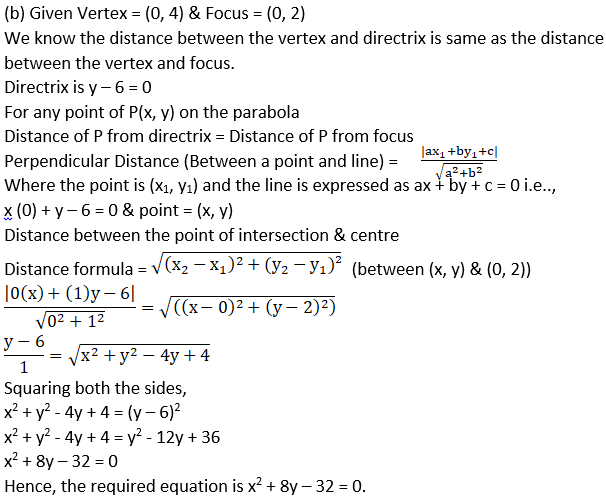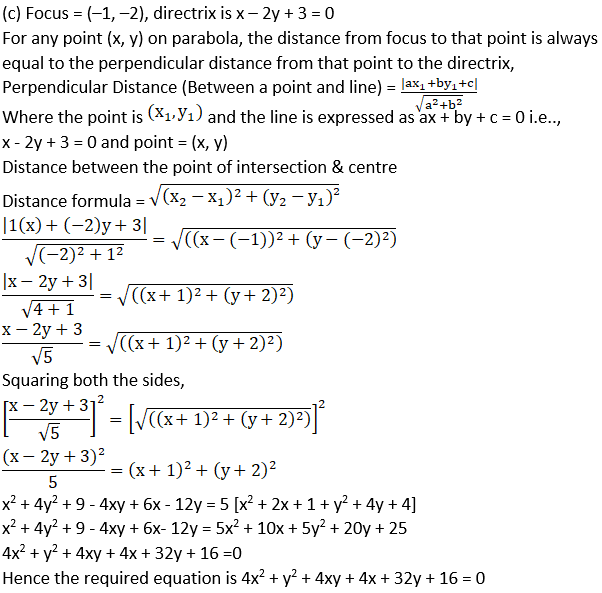# NCERT Exemplar Solutions for Class 11 Maths Chapter 11 Conic Sections

NCERT Exemplar Solutions for Class 11 Maths Chapter 11 Conic Sections are important study resources available for students. NCERT Exemplar books provide multiple-choice questions and very short answer types of questions by which they can boost their problem-solving and time management skills. They have proven to be essential for students to enhance conceptual knowledge and build the confidence that is required to face exams. These questions help them understand the types of questions that will be asked in the final exam. Solutions to all Conic Sections exercise problems help students in preparing for the exam in a better way. The NCERT Exemplar solutions available at BYJU’S will aid them to ace their exams by preparing them well in advance.

Chapter 11 of NCERT Exemplar Solutions for Class 11 Maths Conic Sections explains parabolas, circles and sections of a cone. The exercise-wise solutions of these topics are available here in PDF format, which can be downloaded easily by the students. These solutions are prepared by subject experts at BYJU’S in order to help them with their exam preparation. Some of the important topics of this chapter are listed below.

• Sections of a cone
• Circle and equation of a circle
• Parabola
• Directrix of the parabola
• Standard equations of parabola
• Focal distance of a point of a parabola
• Ellipse
• Focal distance of an ellipse
• Hyperbola
• Focal distance of hyperbola
• Parametric equation of conics

## Download the PDF of the NCERT Exemplar Solutions for Class 11 Maths Chapter 11 Conic Sections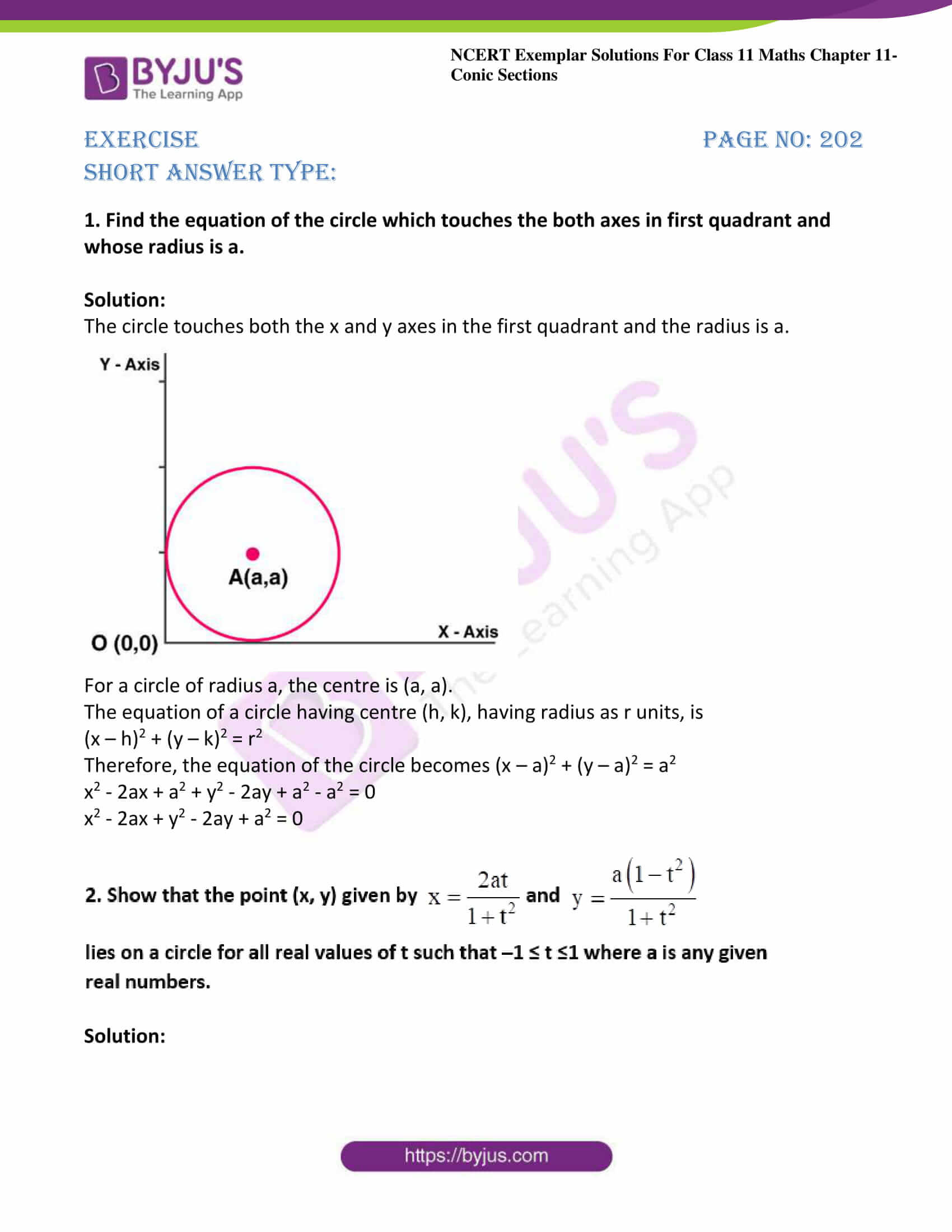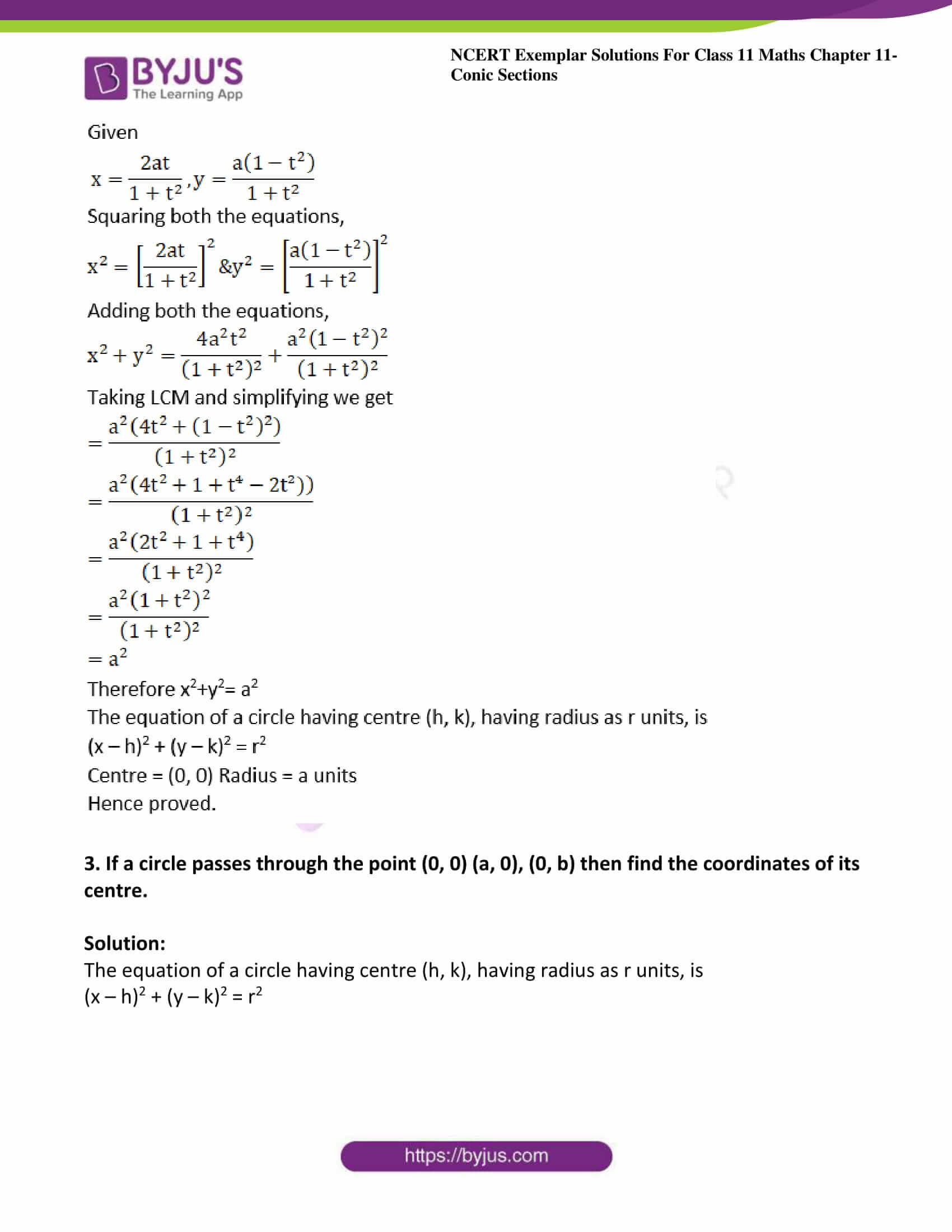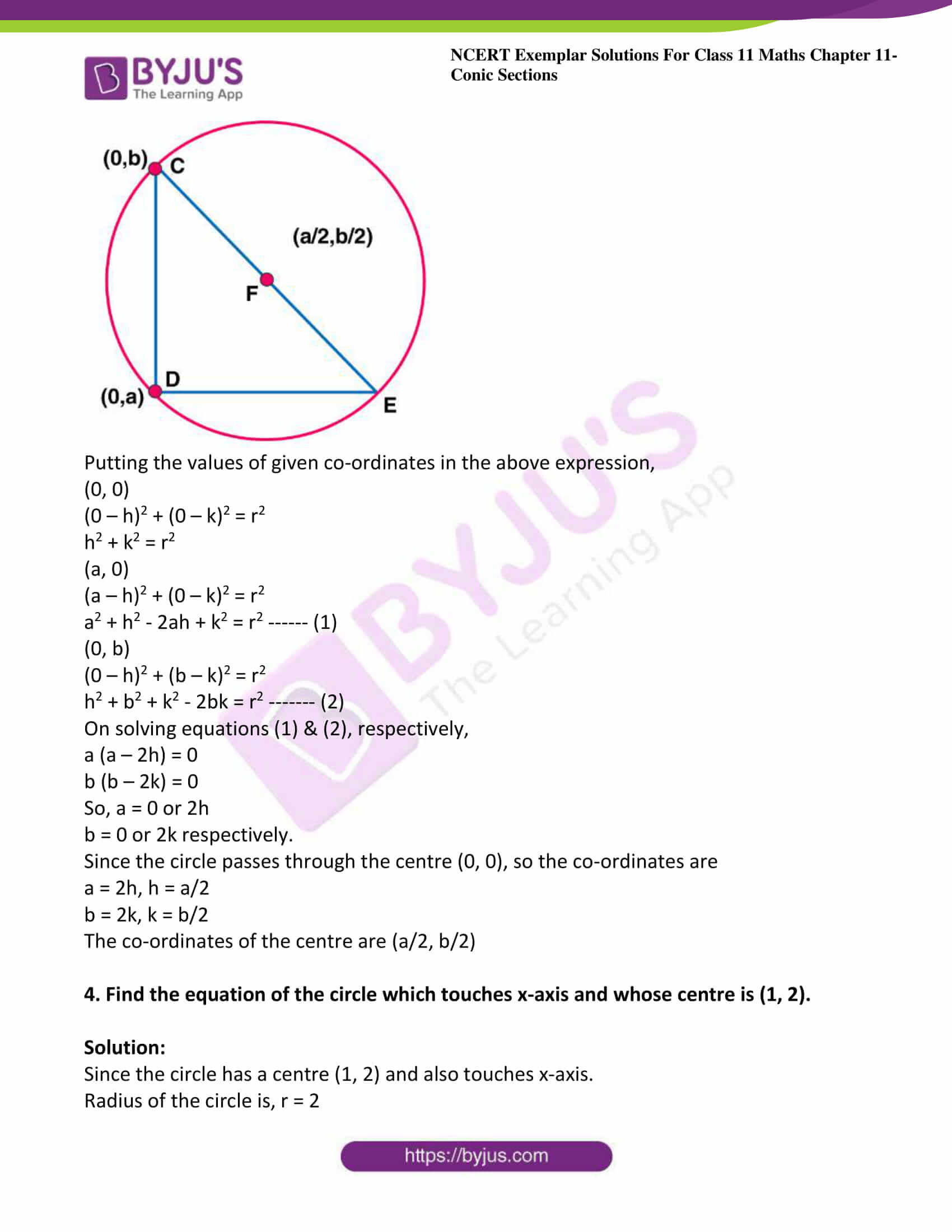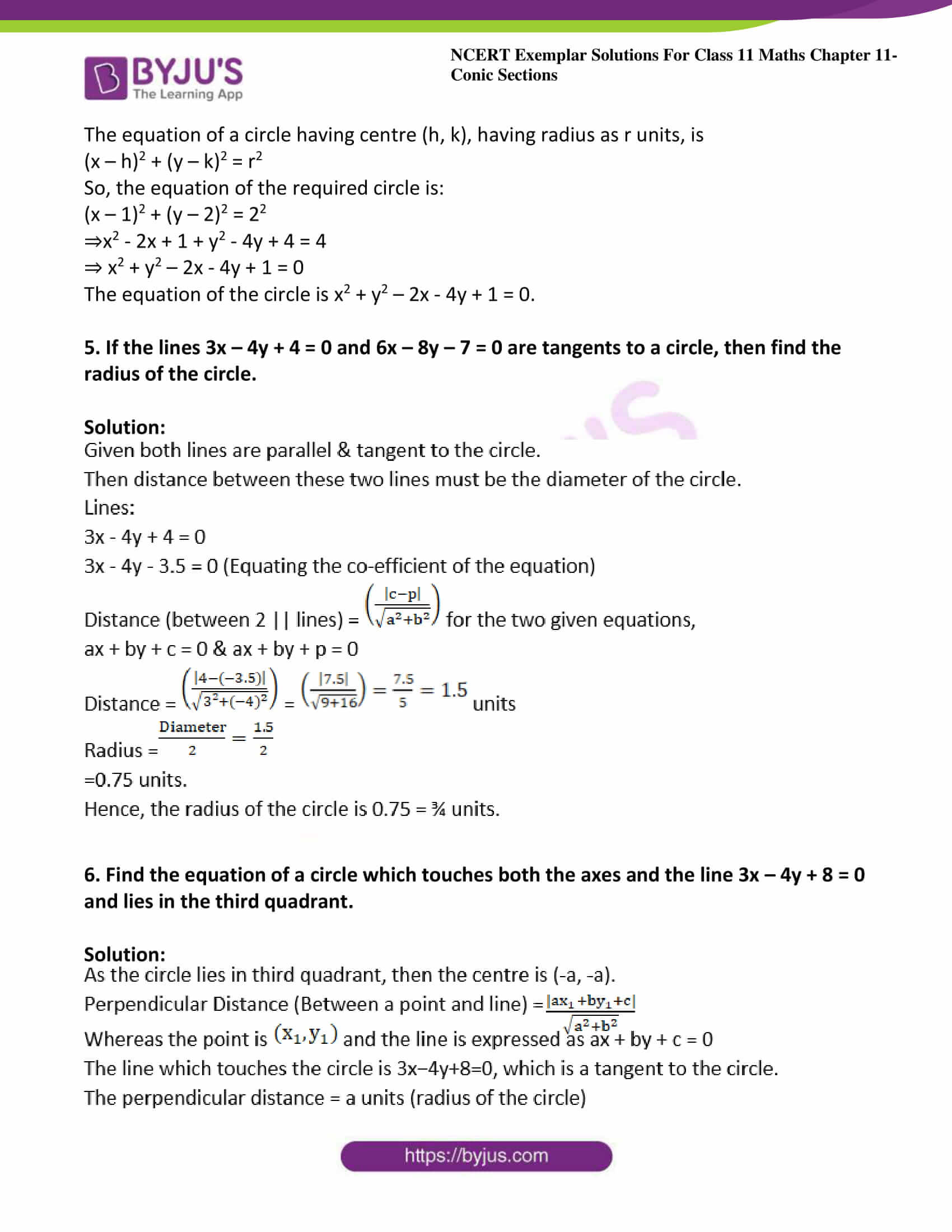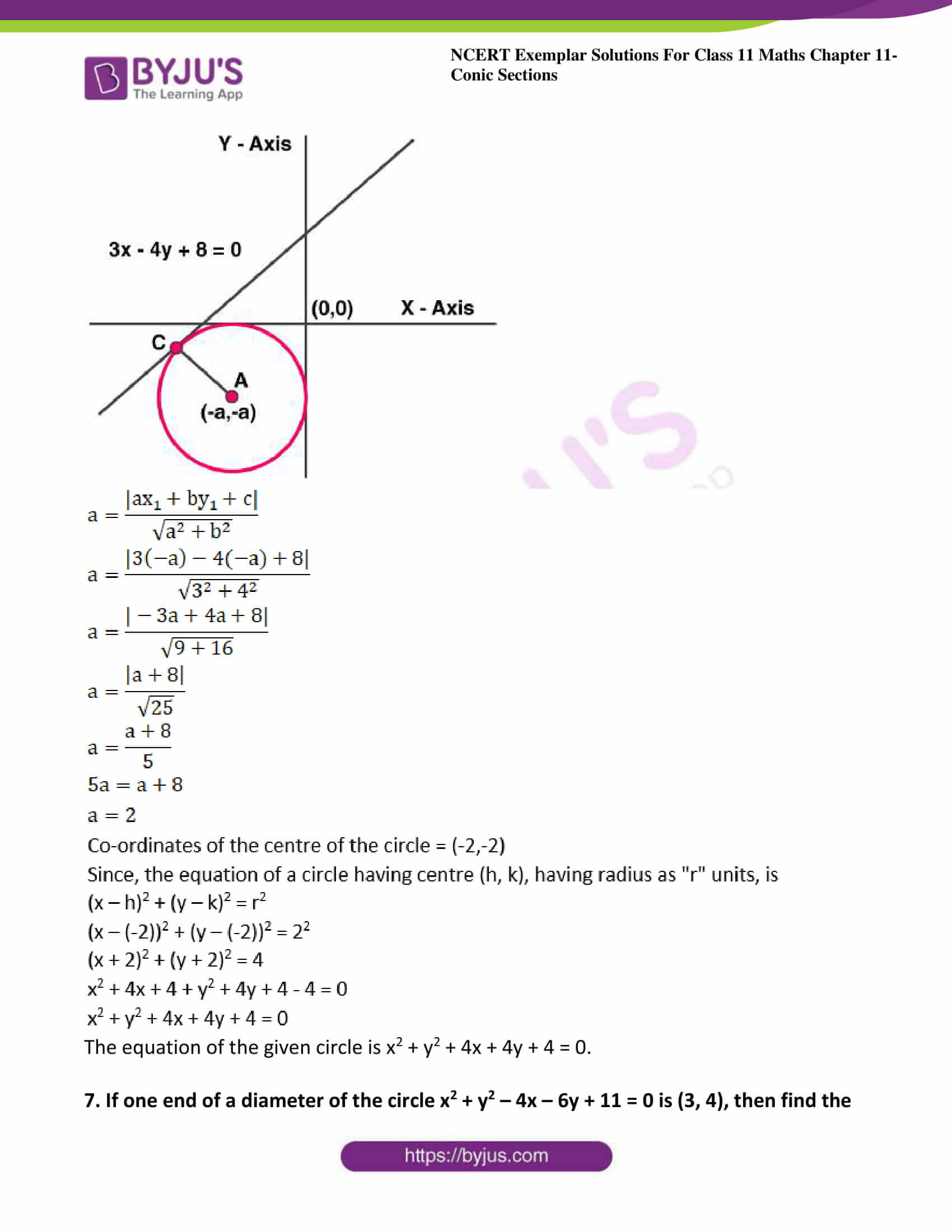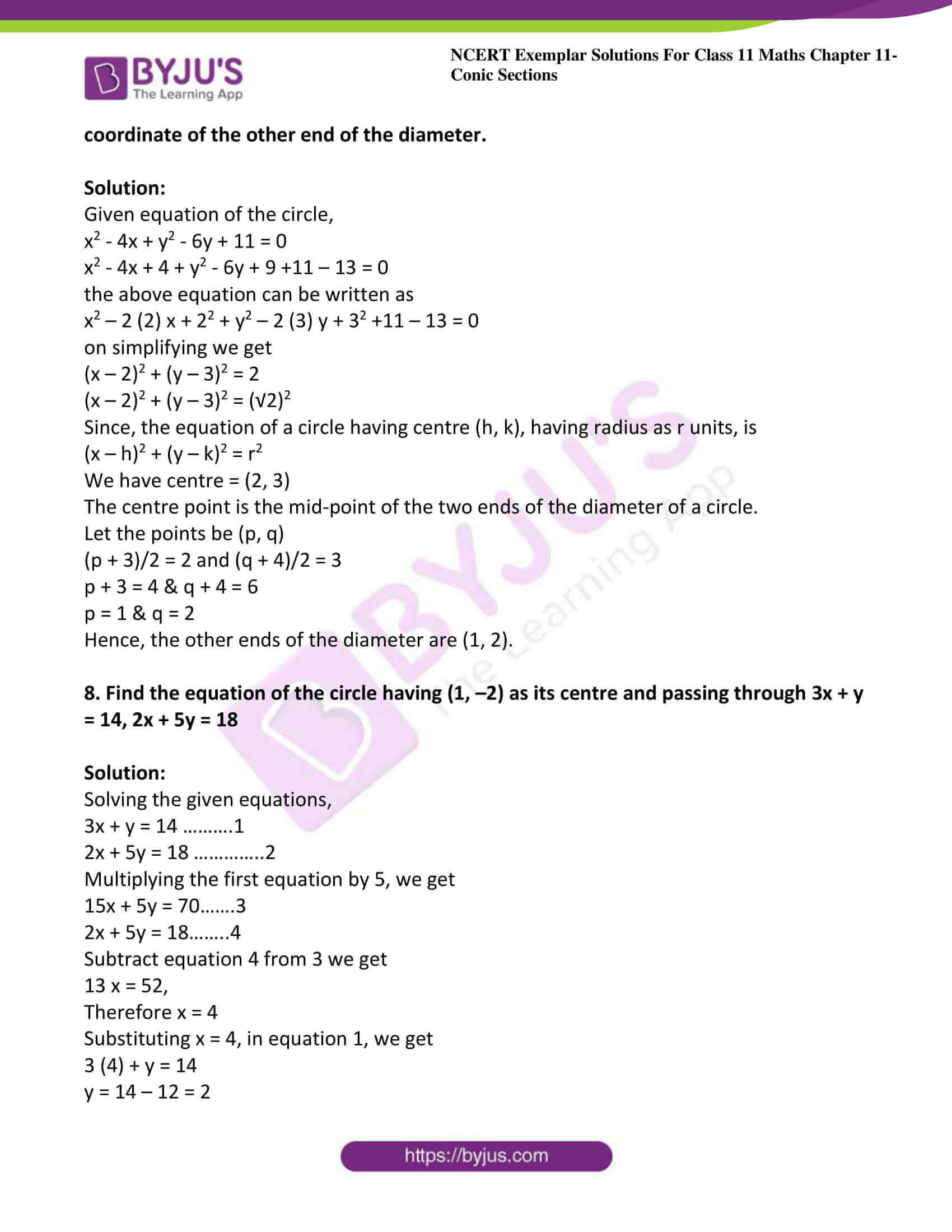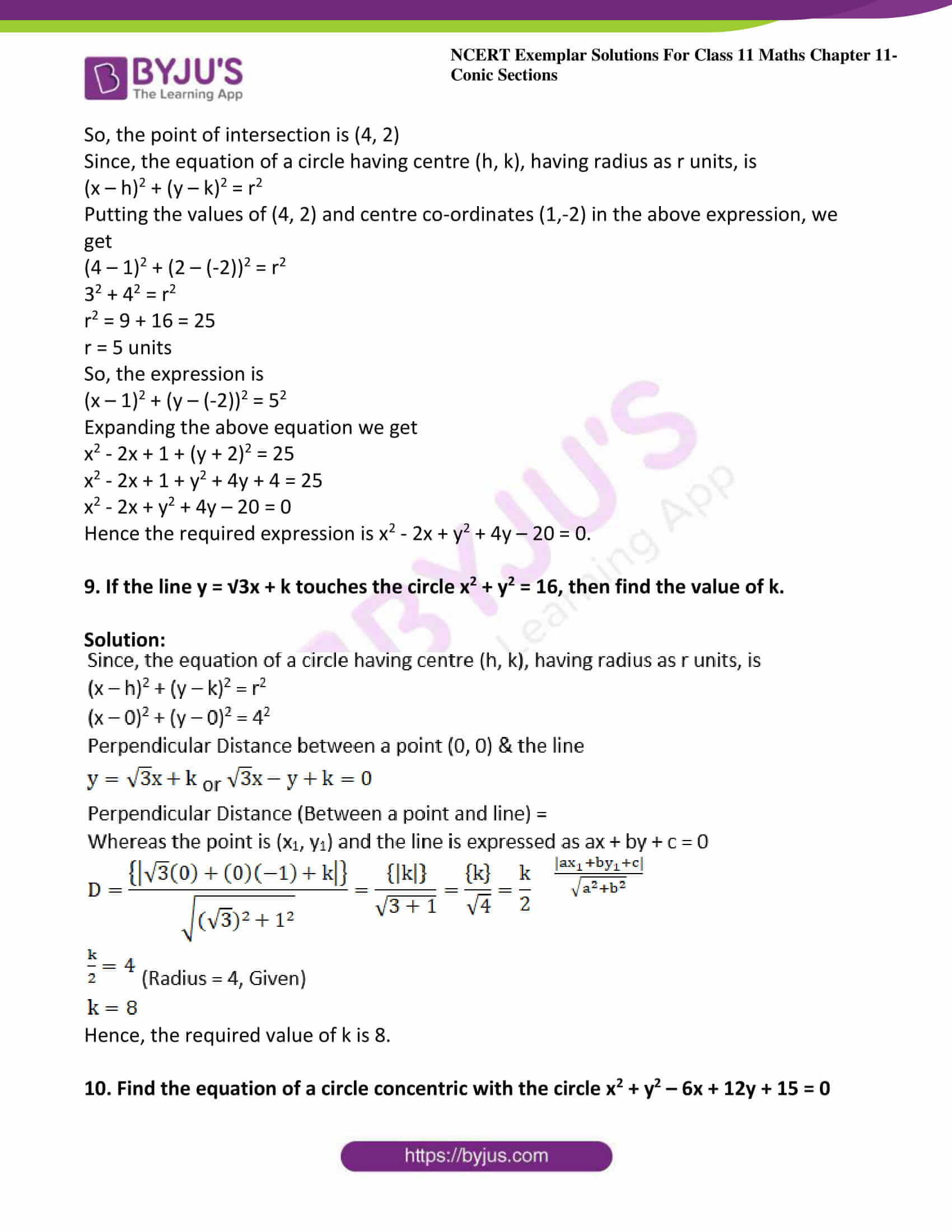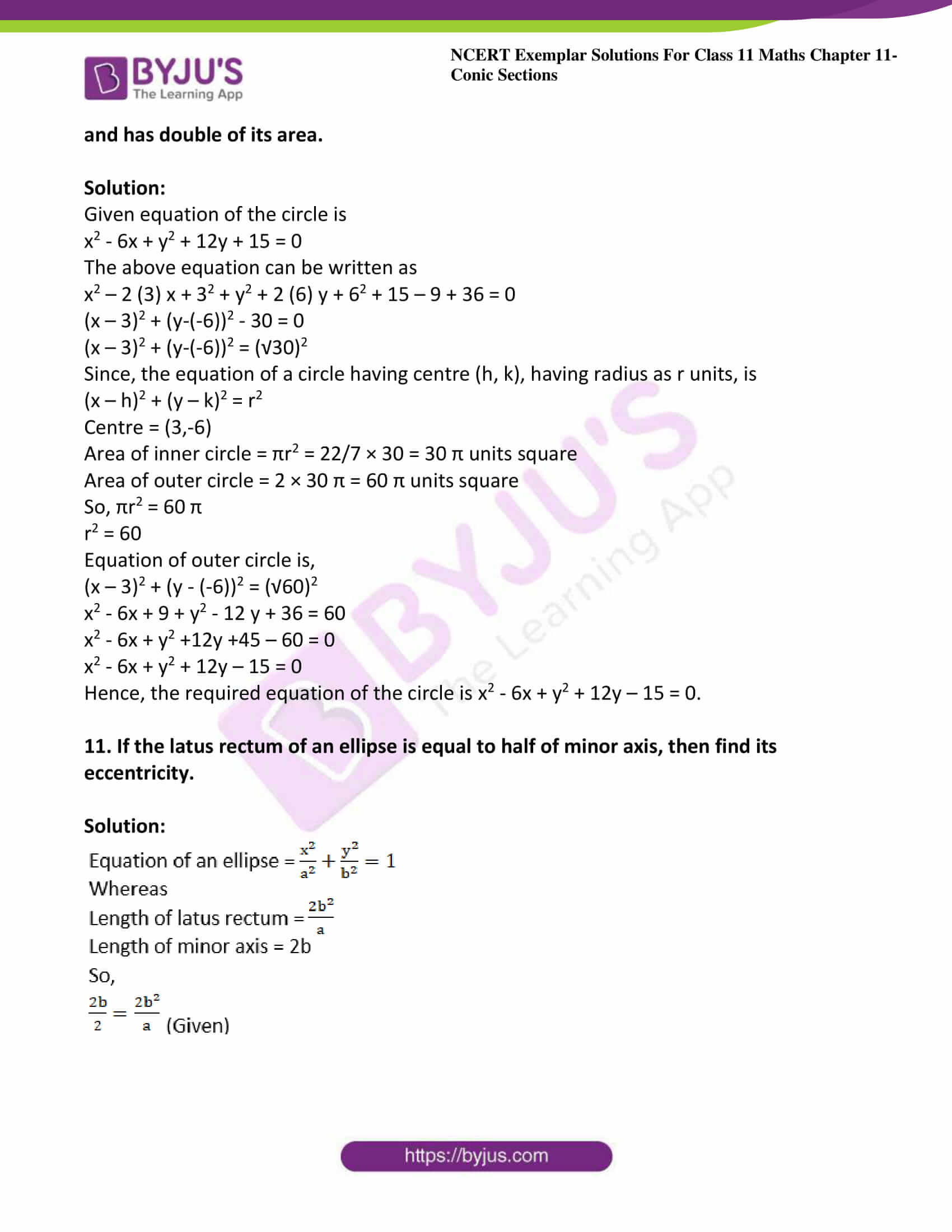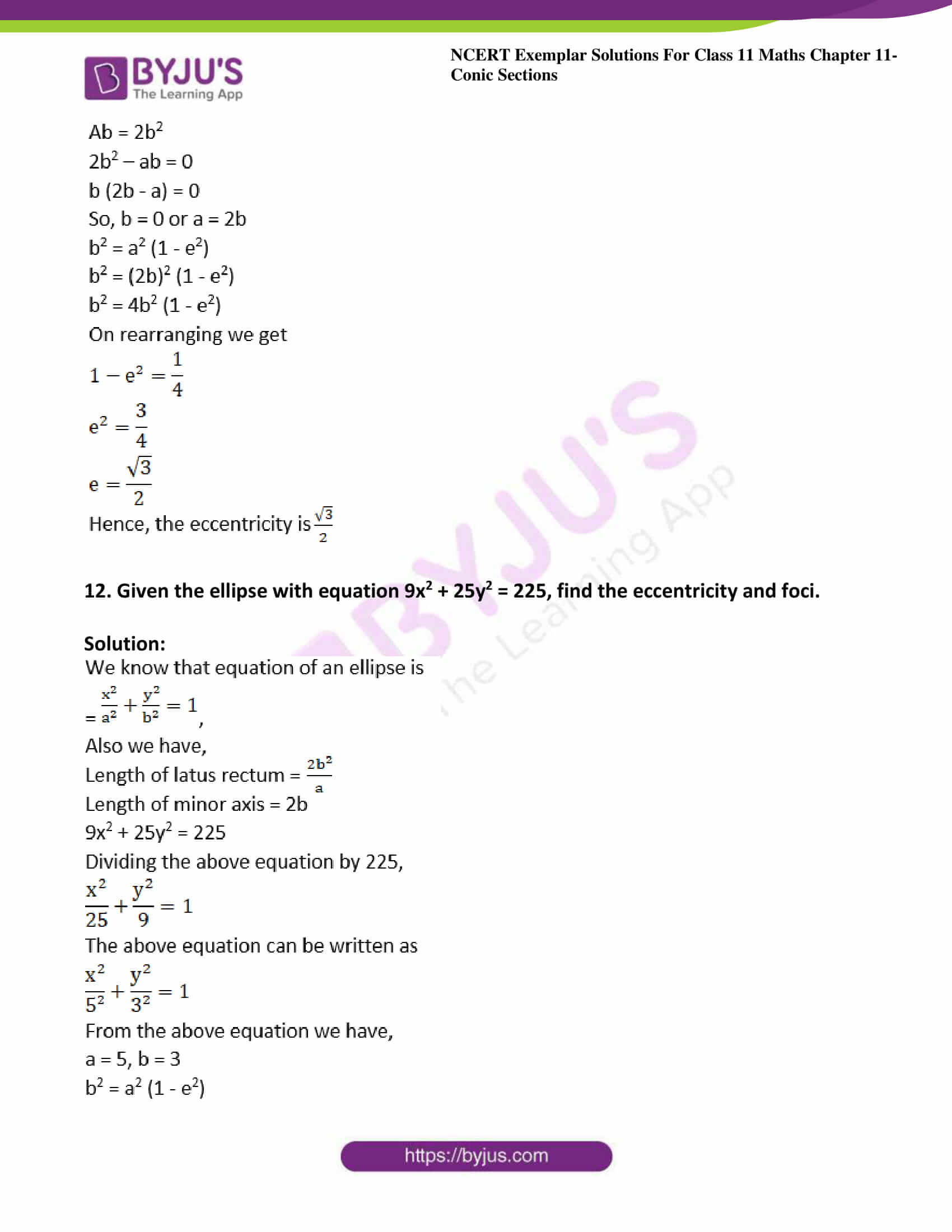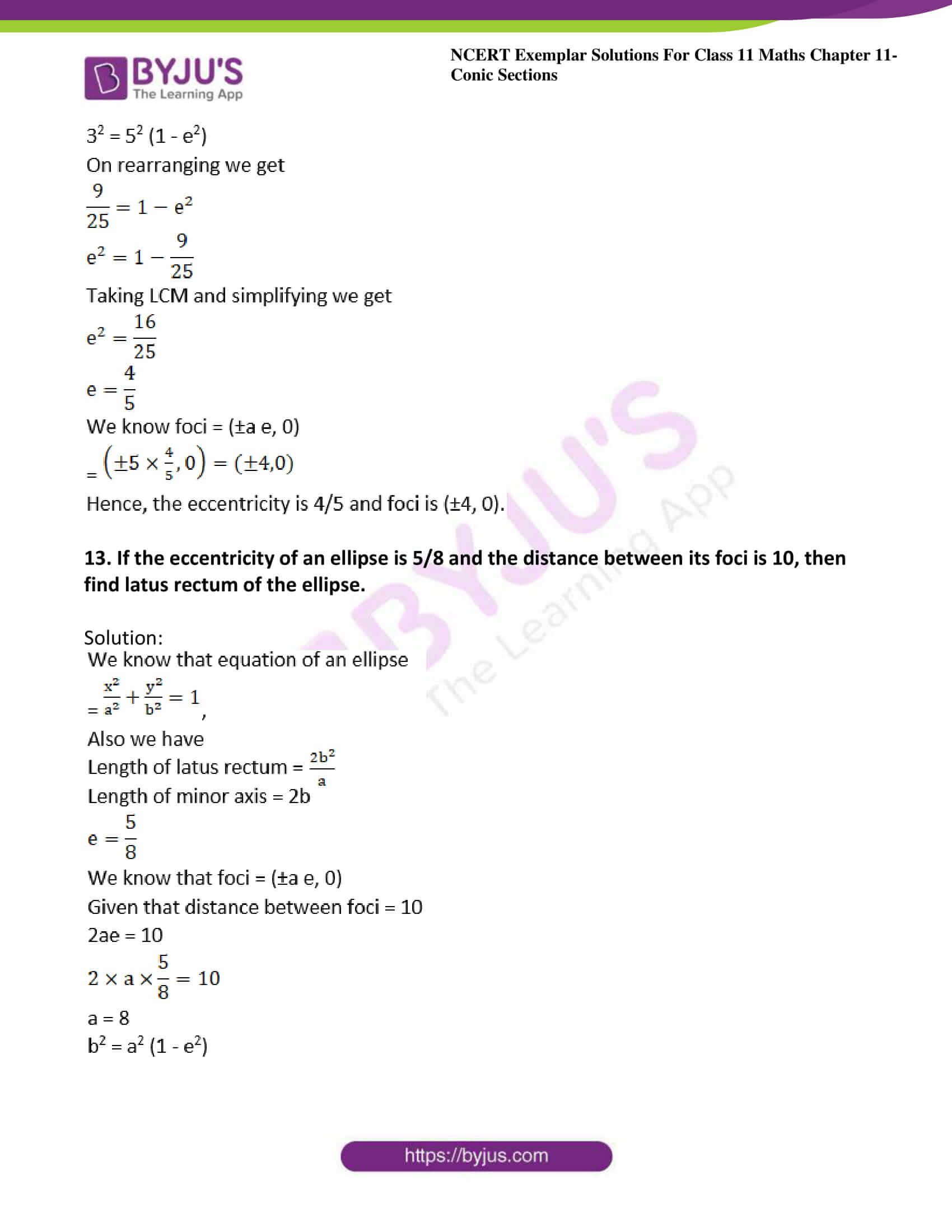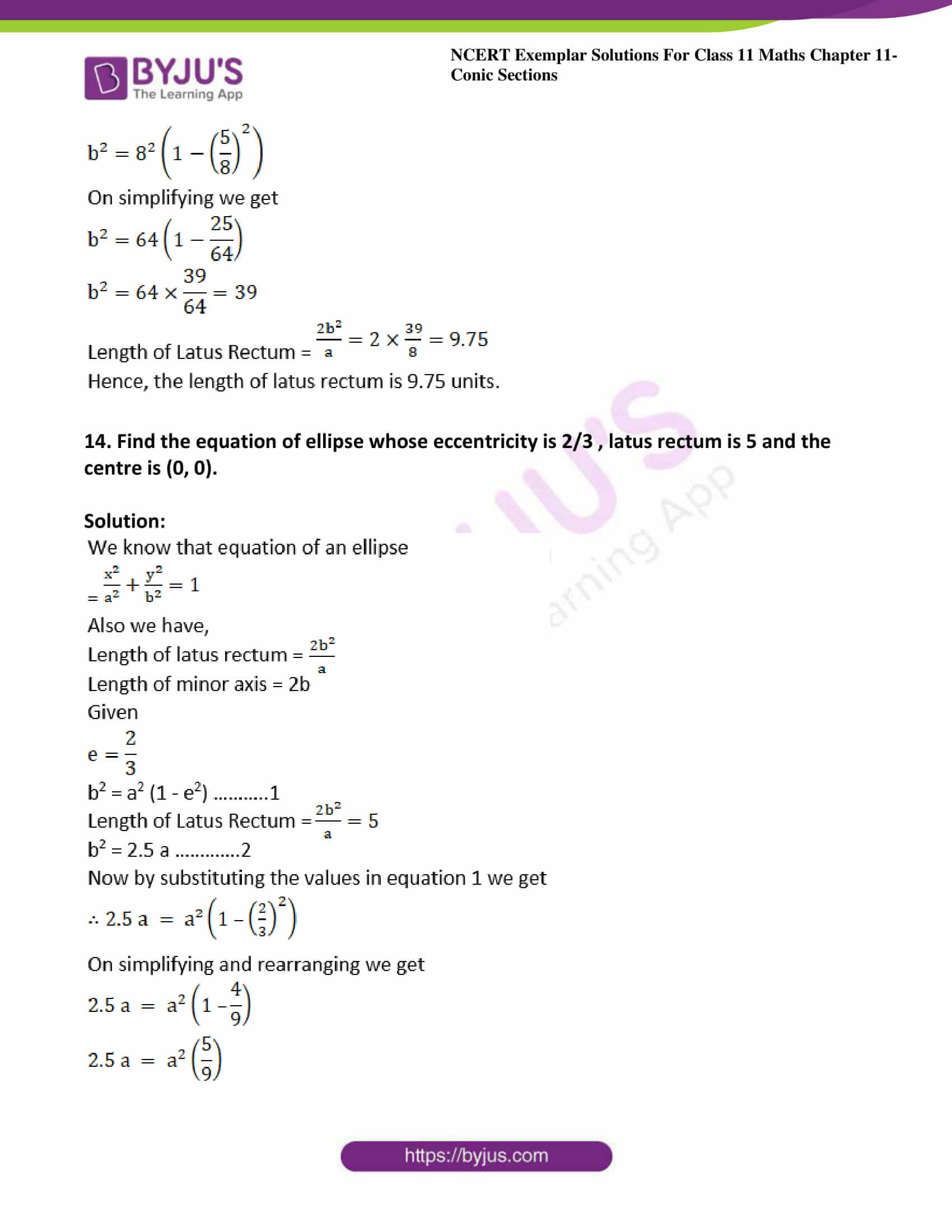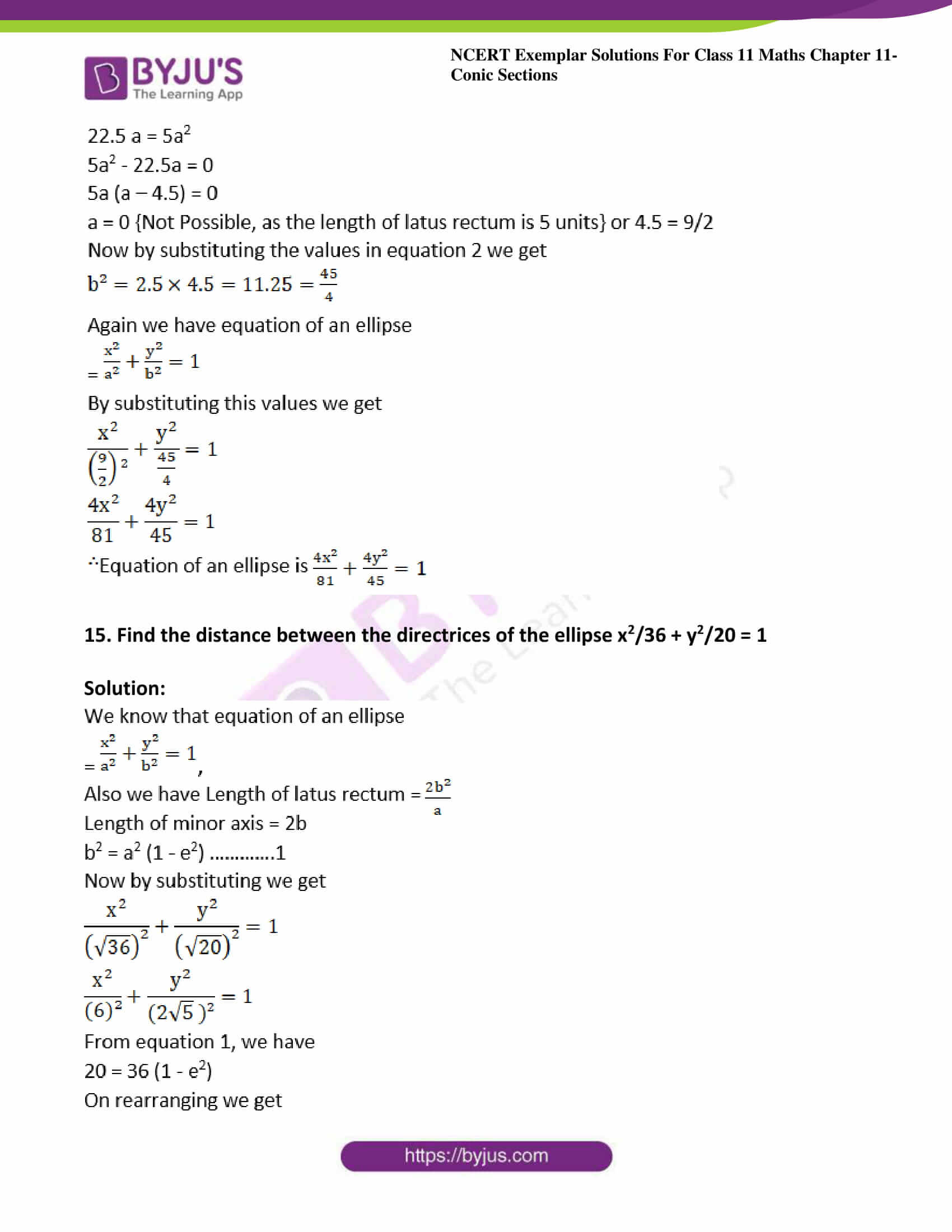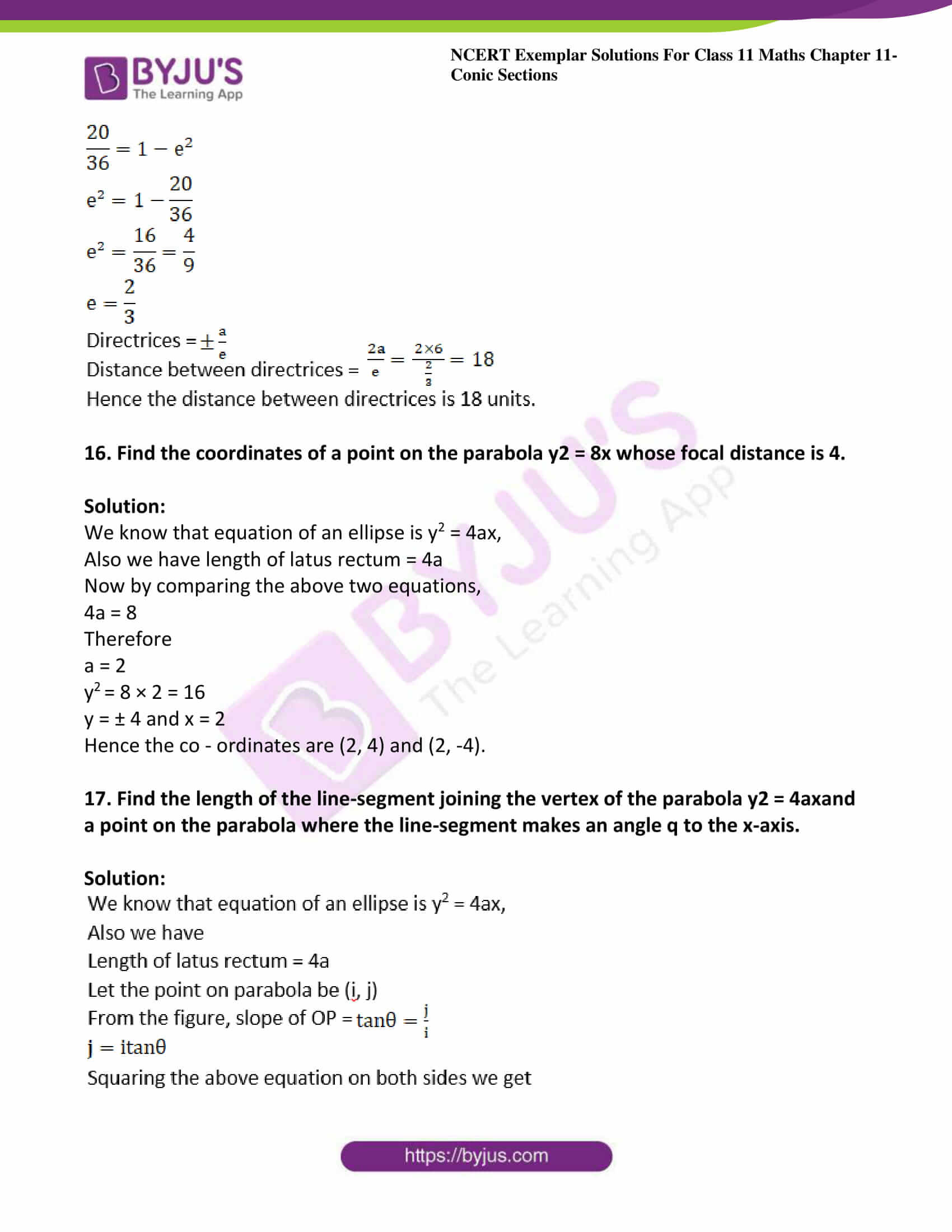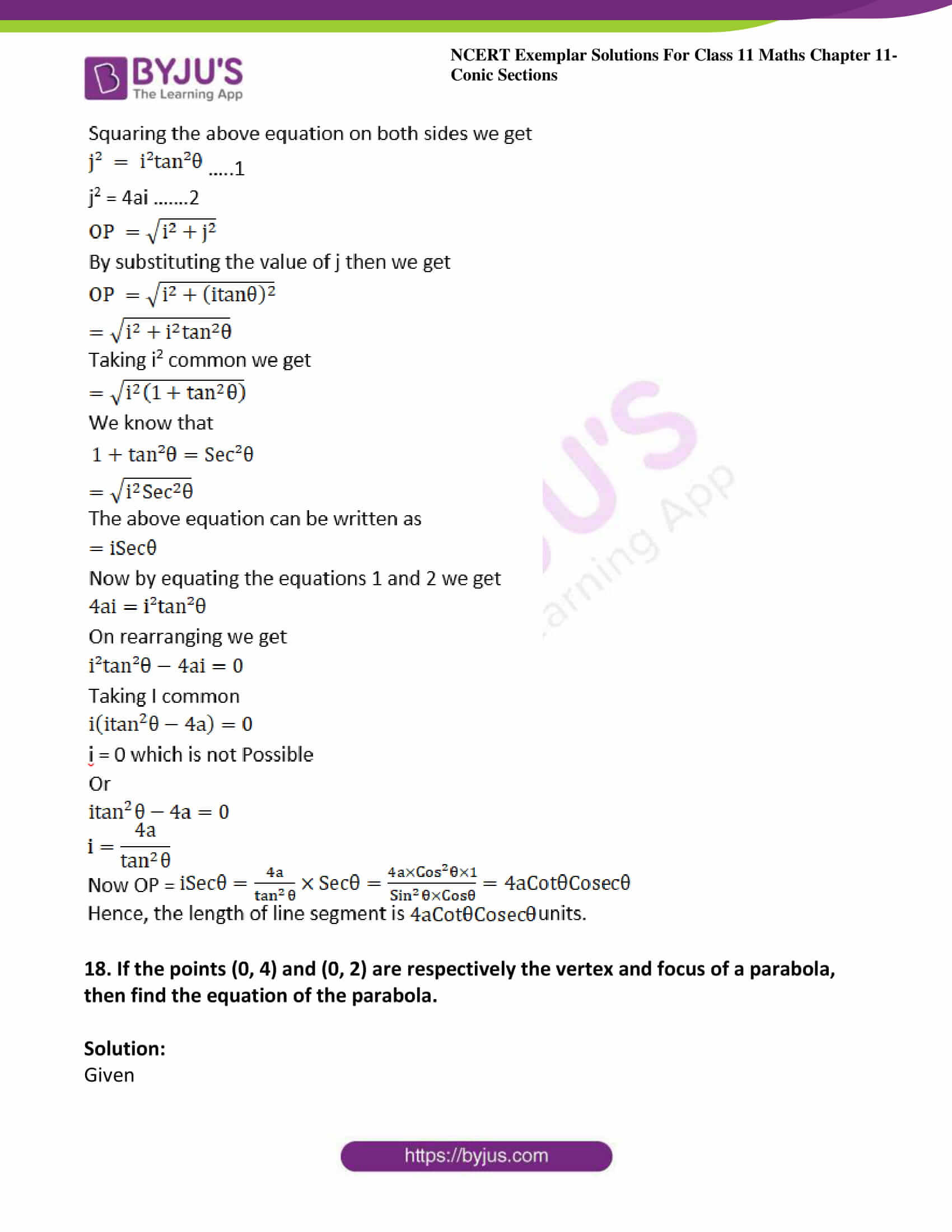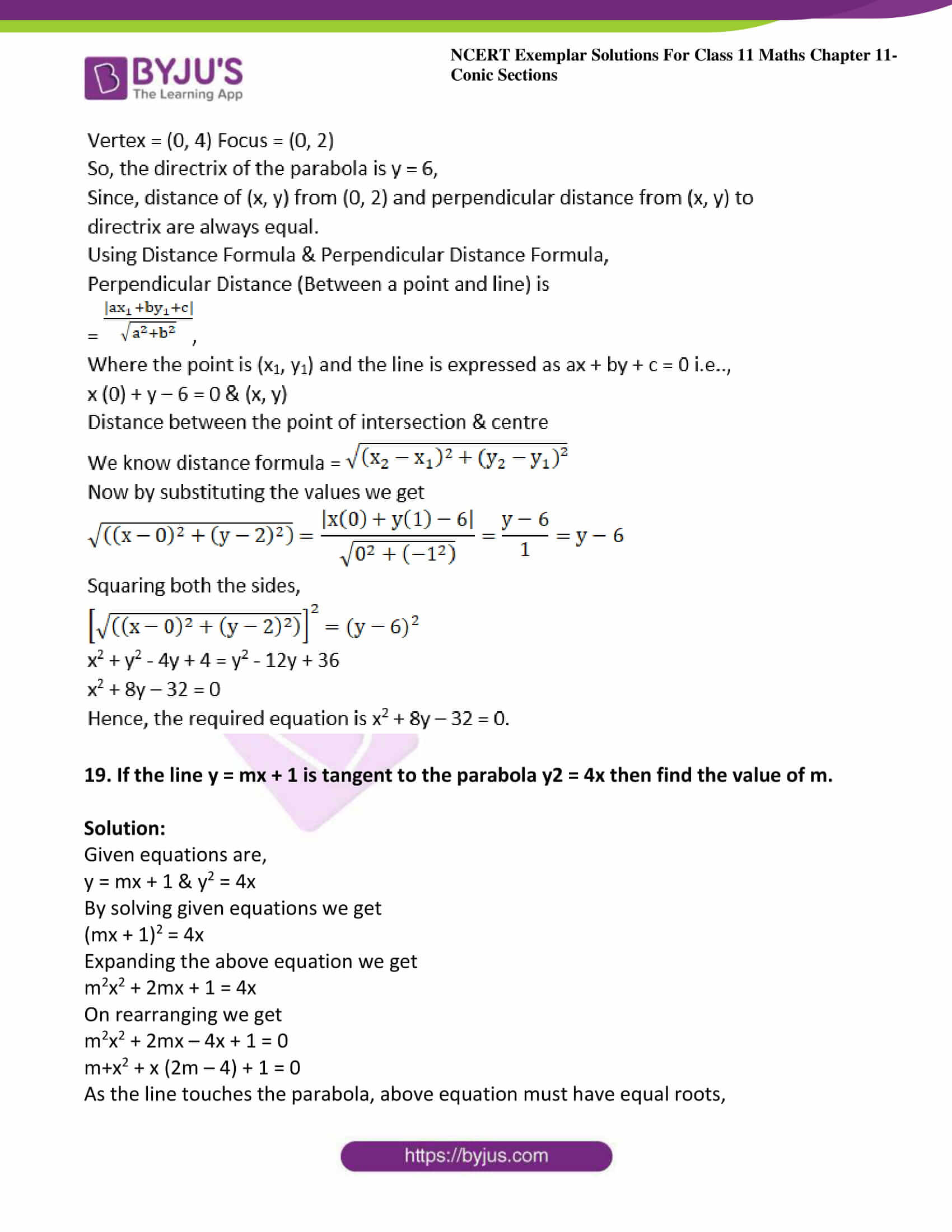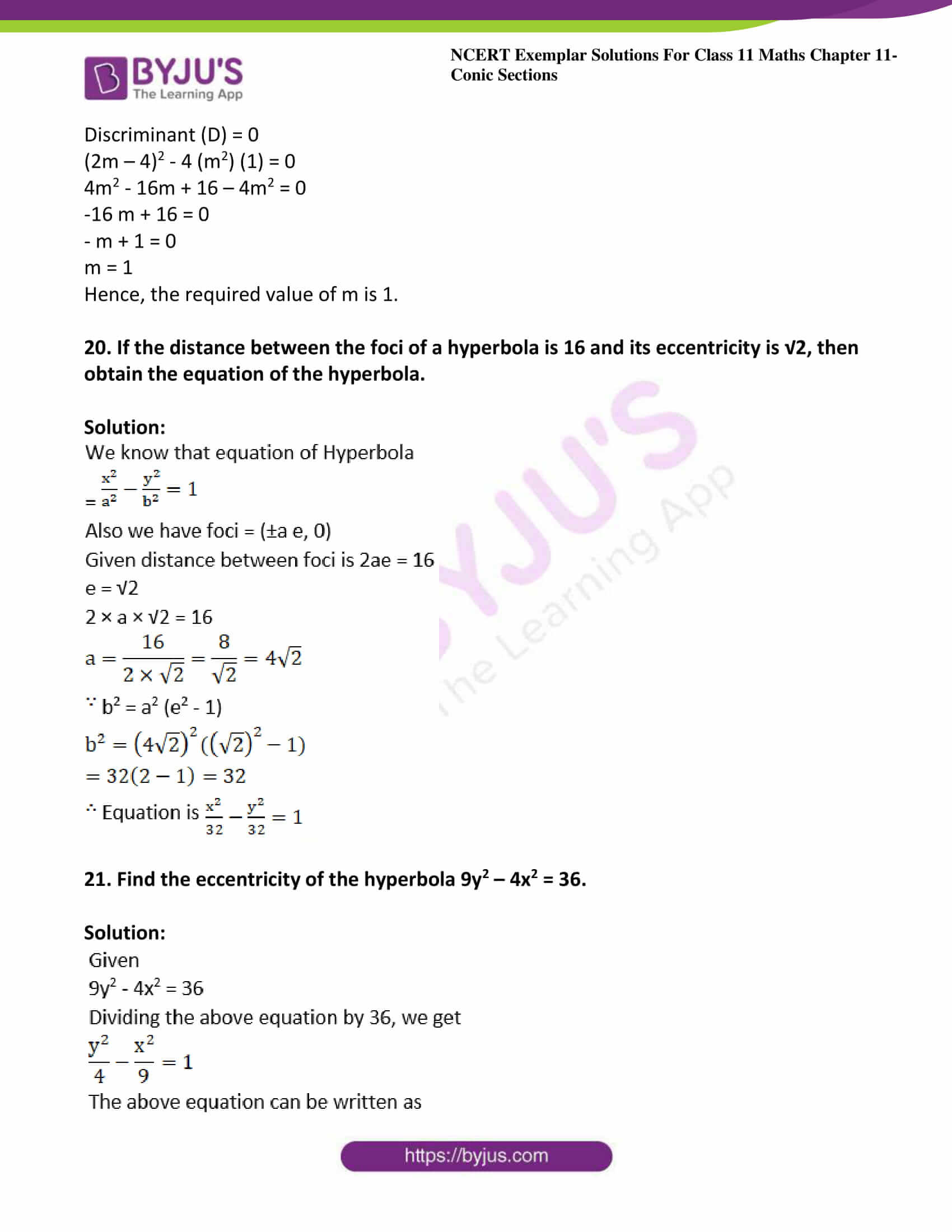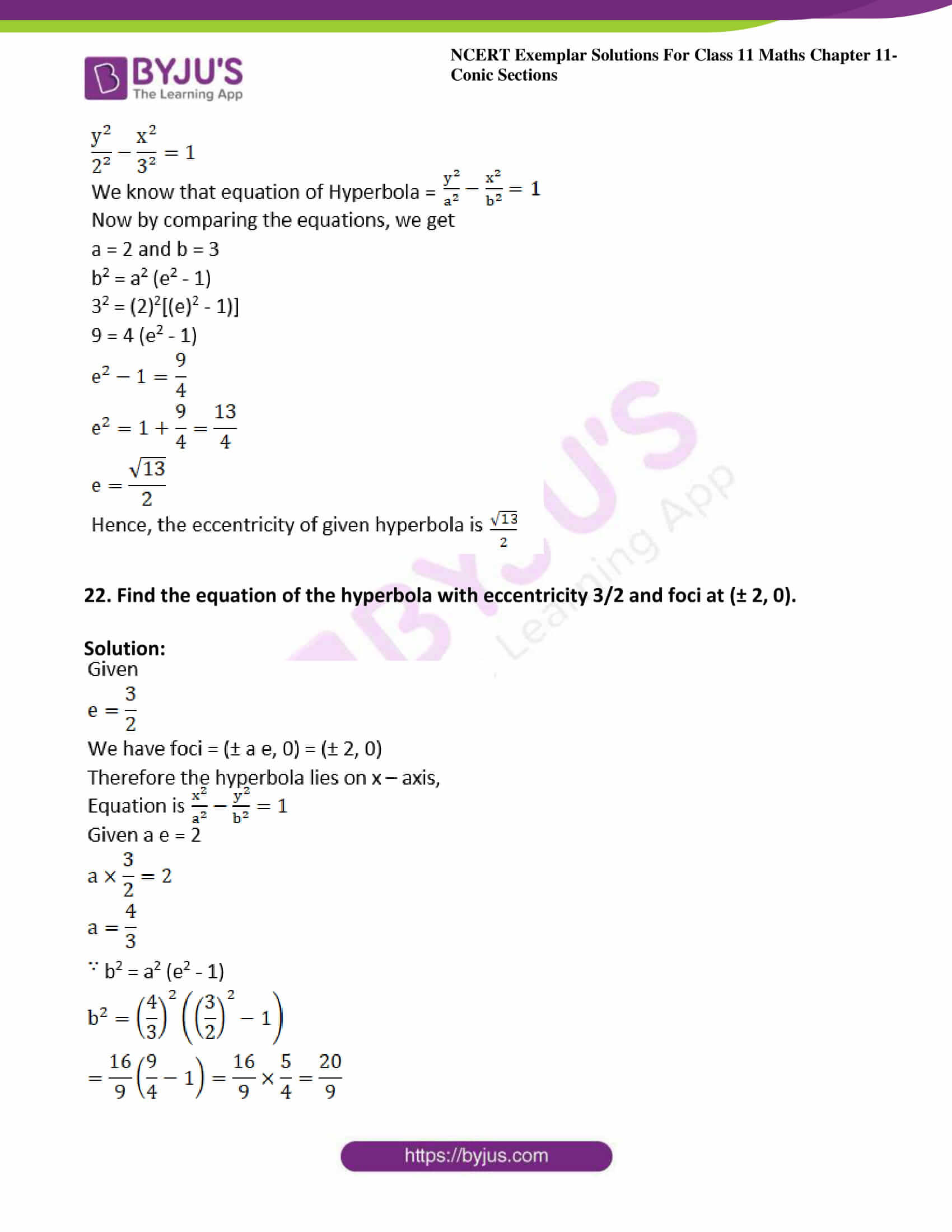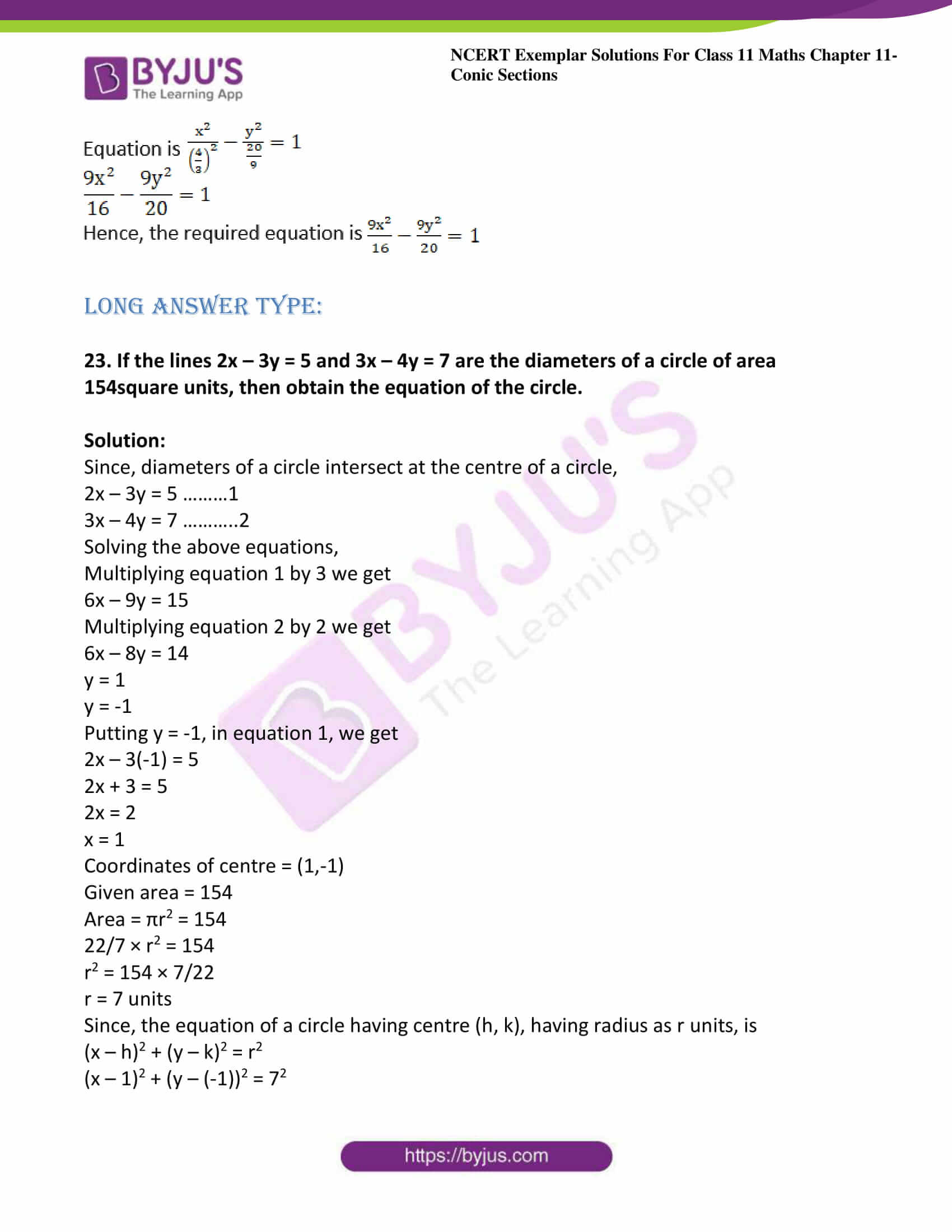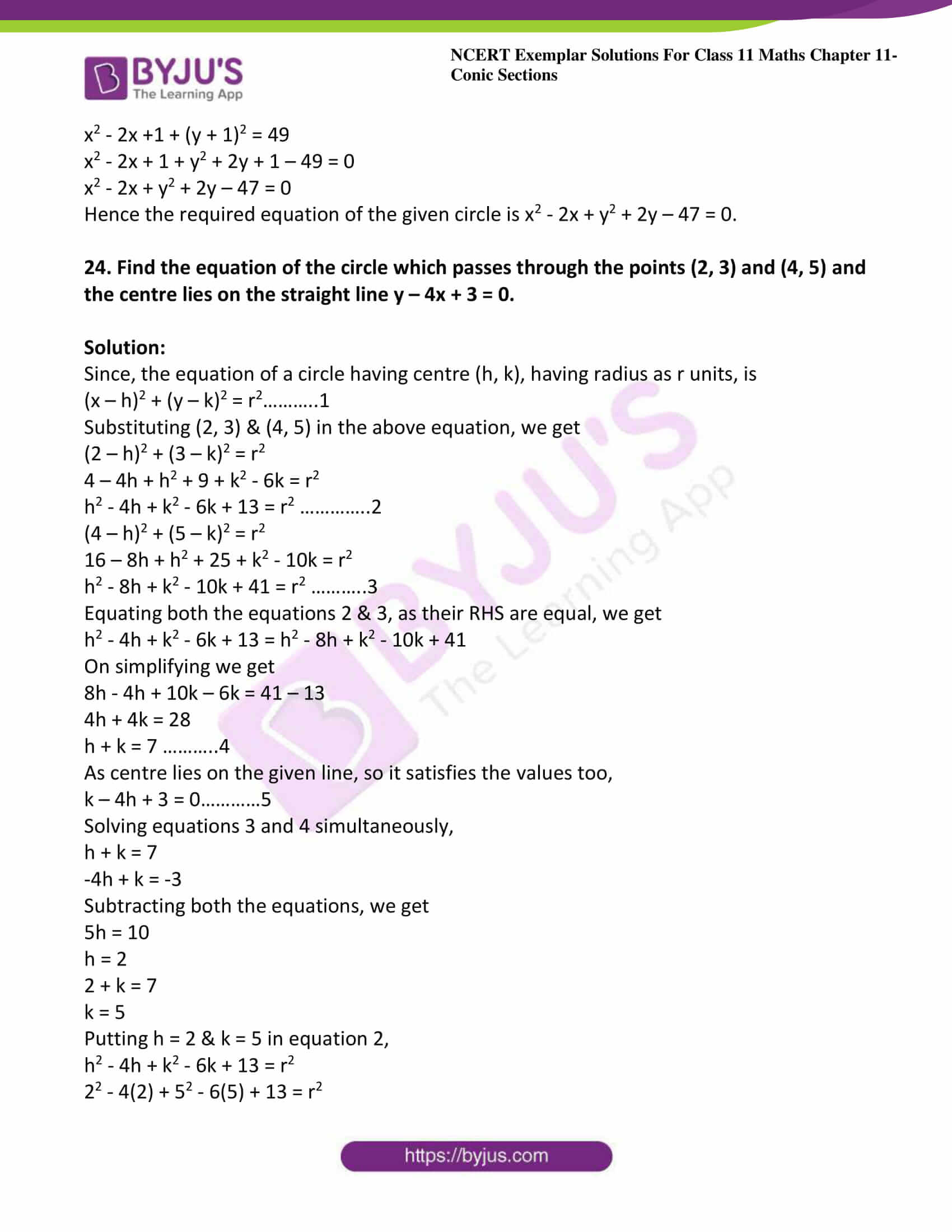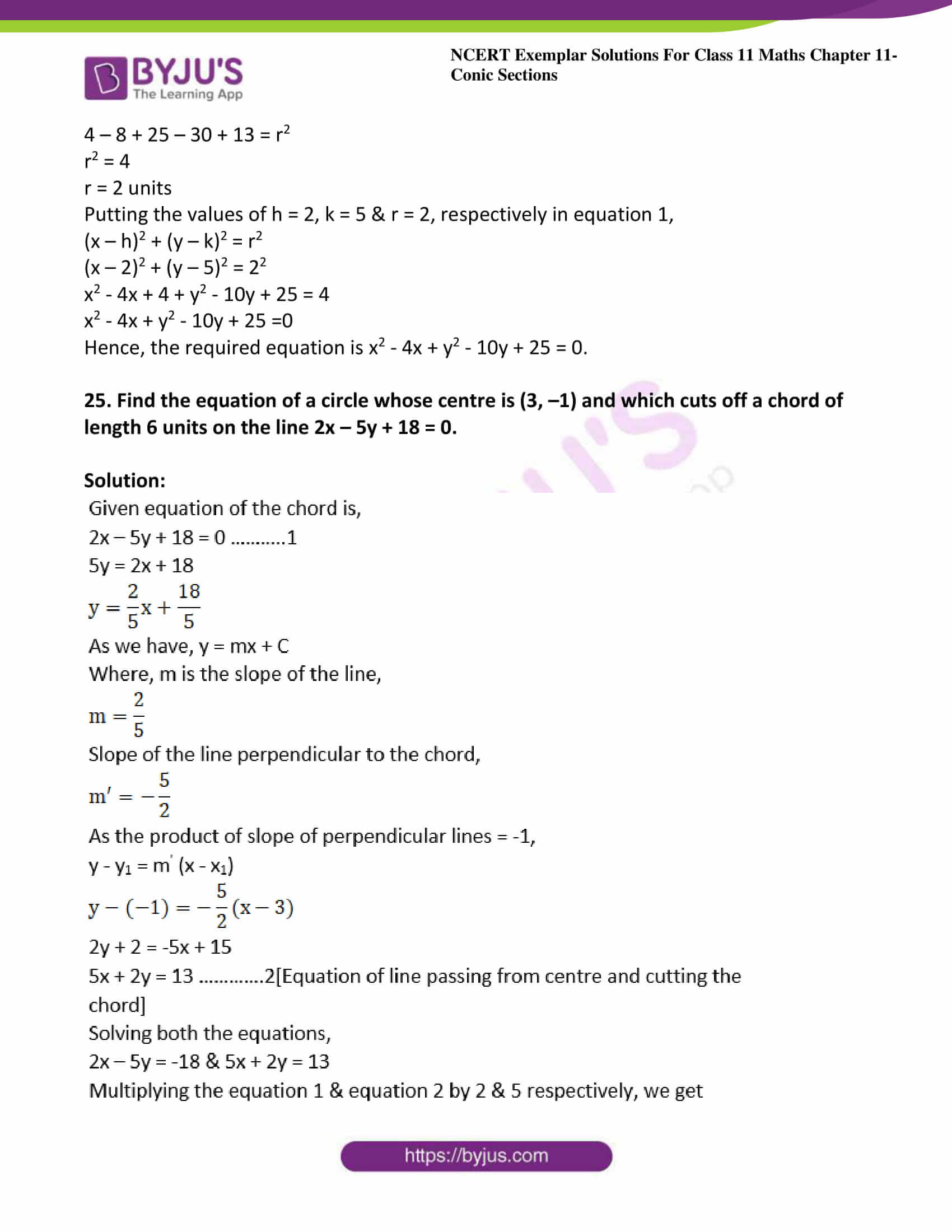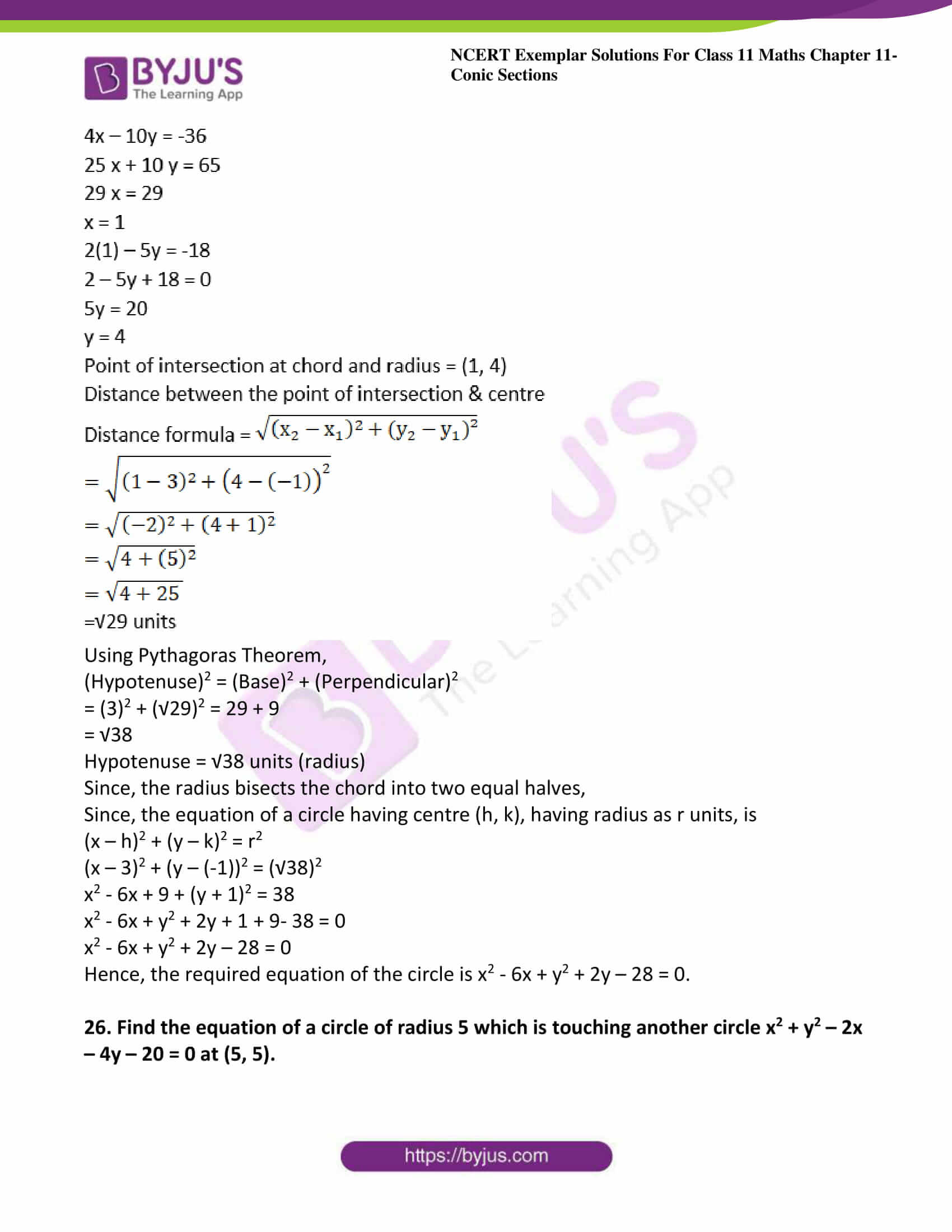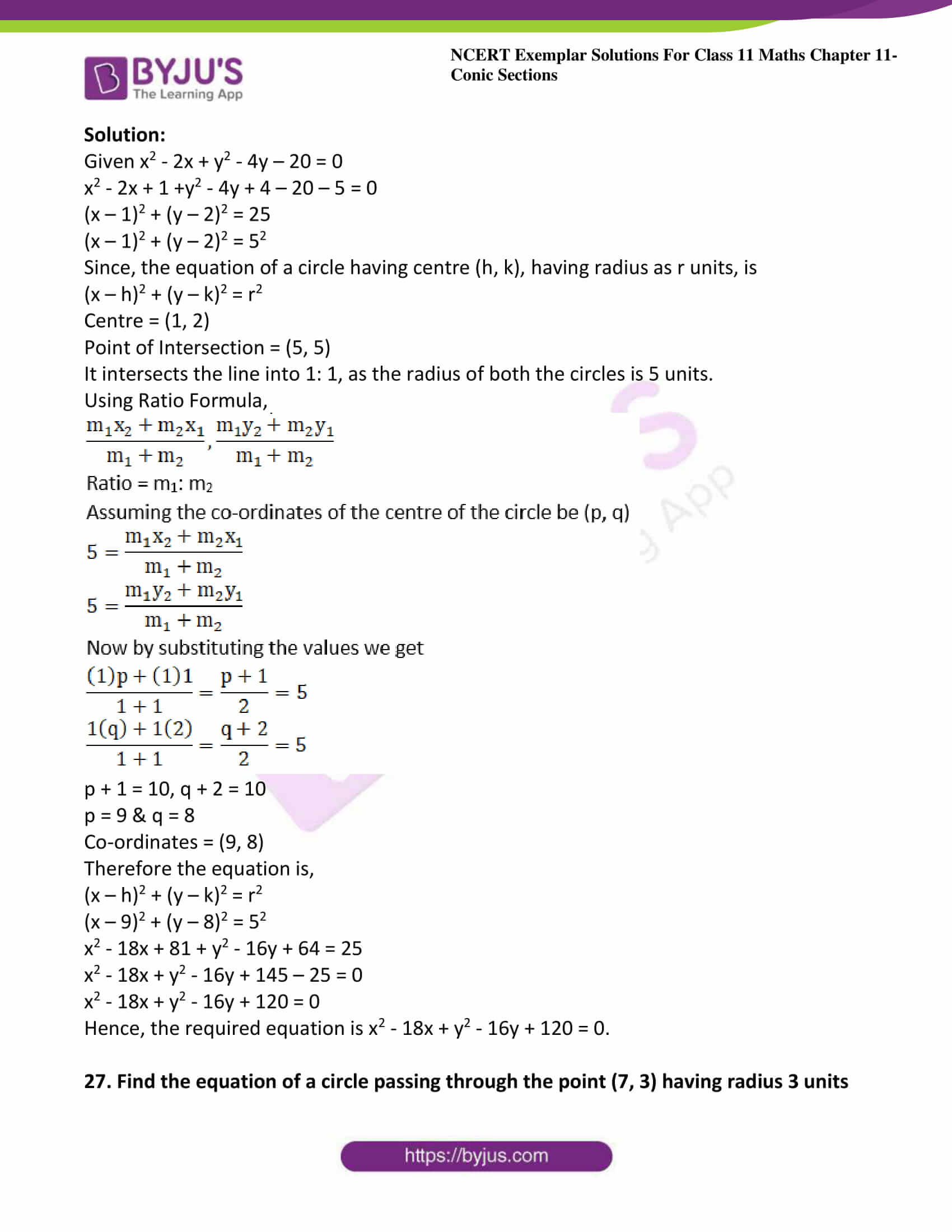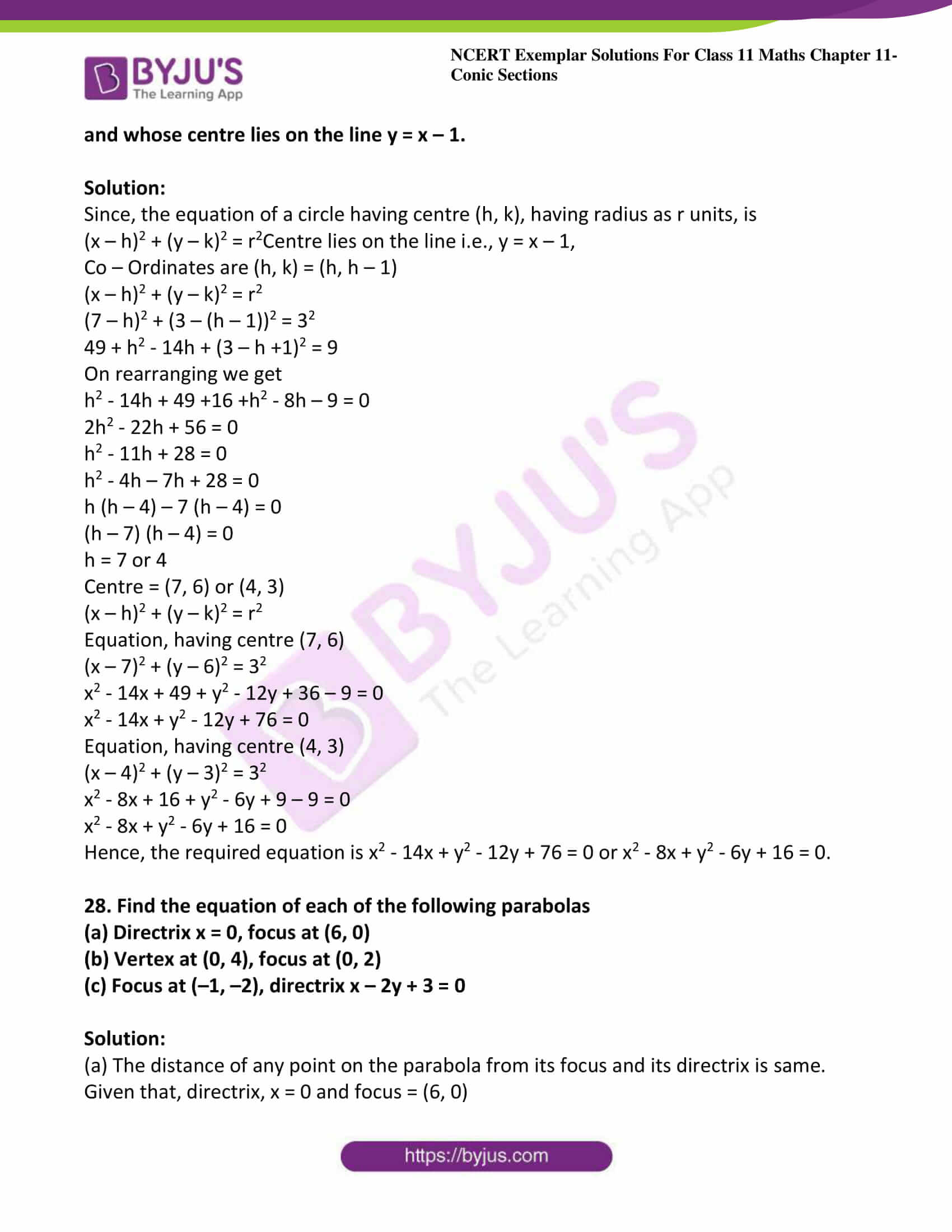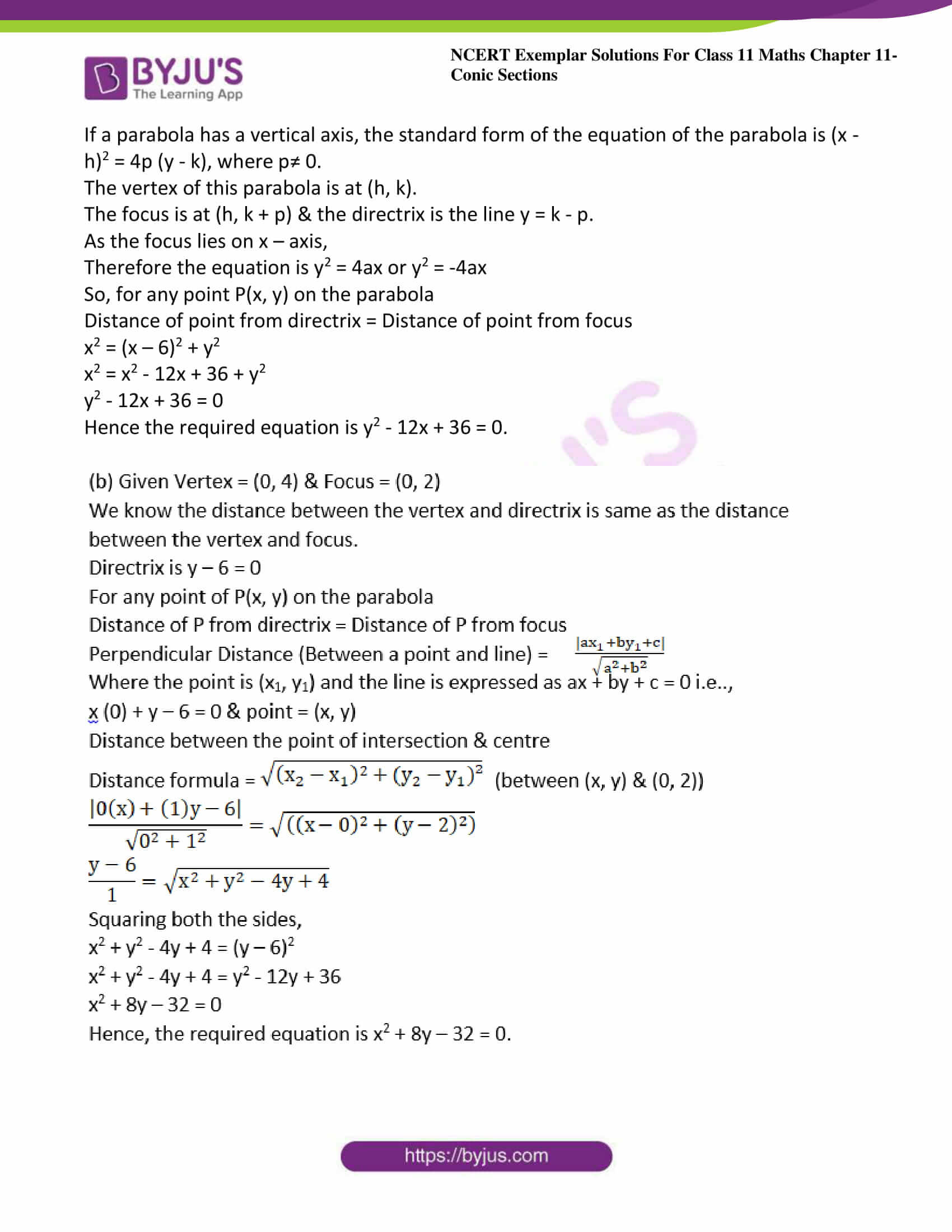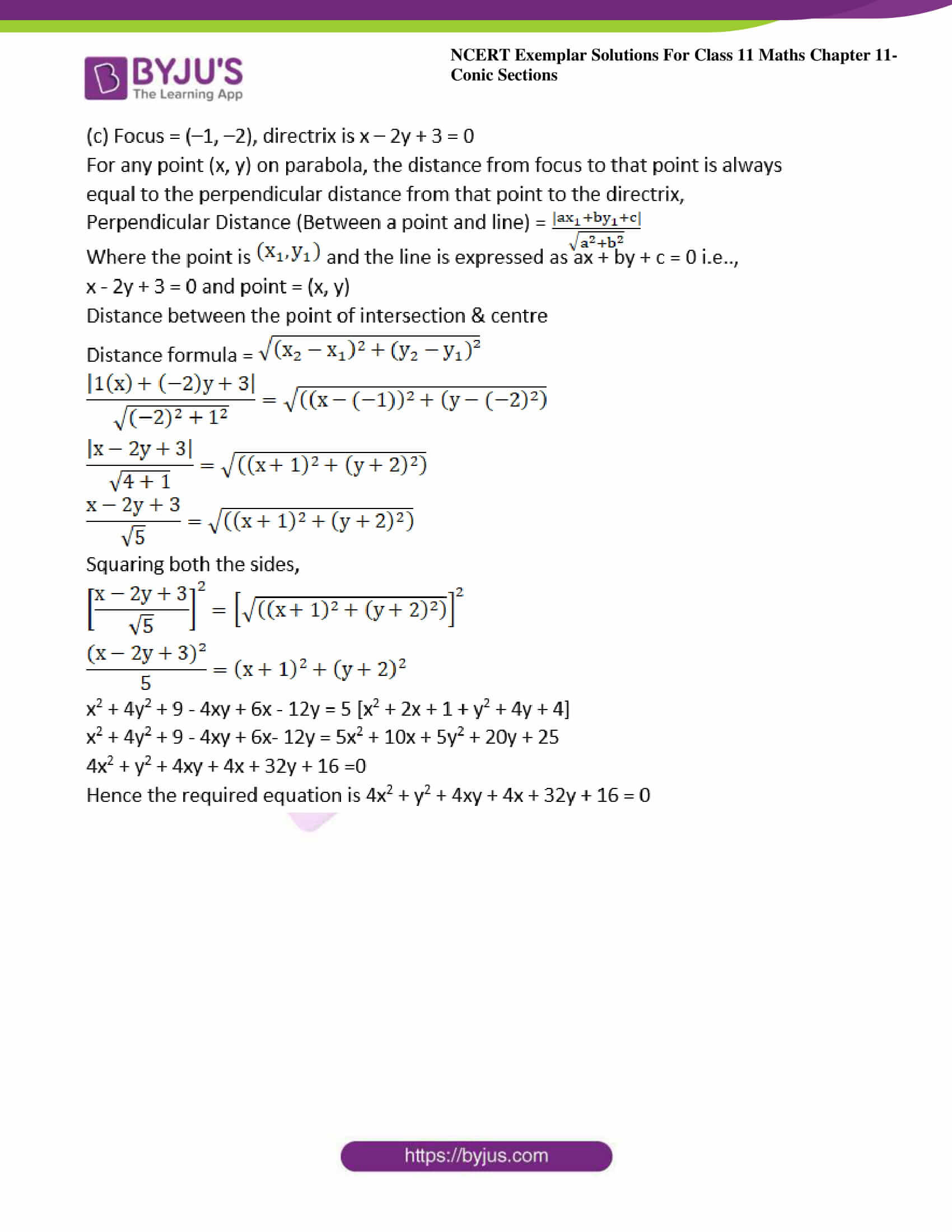### Access Answers to NCERT Exemplar Solutions for Class 11 Maths Chapter 11 – Conic Sections

Exercise Page No: 202

1. Find the equation of the circle which touches the both axes in first quadrant and whose radius is a.

Solution:

The circle touches both the x and y axes in the first quadrant and the radius is a.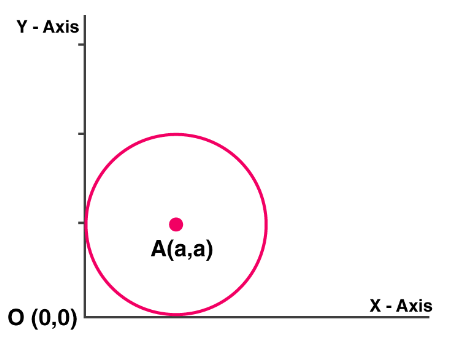For a circle of radius a, the centre is (a, a).

The equation of a circle having centre (h, k), having radius as r units, is

(x – h)2 + (y – k)2 = r2

Therefore, the equation of the circle becomes (x – a)2 + (y – a)2 = a2

x2 – 2ax + a2 + y2 – 2ay + a2 – a2 = 0

x2 – 2ax + y2 – 2ay + a2 = 0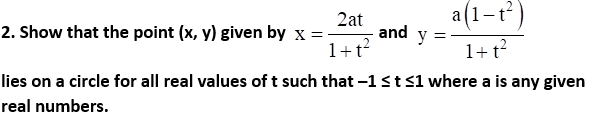Solution: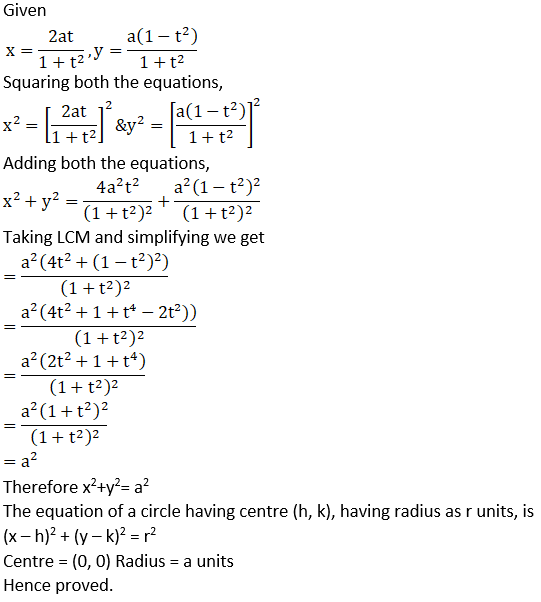3. If a circle passes through the point (0, 0) (a, 0), (0, b) then find the coordinates of its centre.

Solution:

The equation of a circle having centre (h, k), having radius as r units, is

(x – h)2 + (y – k)2 = r2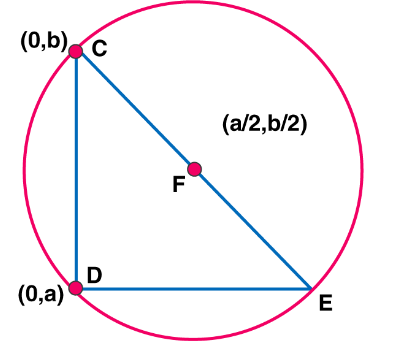Putting the values of given co-ordinates in the above expression,

(0, 0)

(0 – h)2 + (0 – k)2 = r2

h2 + k2 = r2

(a, 0)

(a – h)2 + (0 – k)2 = r2

a2 + h2 – 2ah + k2 = r2 —— (1)

(0, b)

(0 – h)2 + (b – k)2 = r2

h2 + b2 + k2 – 2bk = r2 ——- (2)

On solving equations (1) & (2), respectively,

a (a – 2h) = 0

b (b – 2k) = 0

So, a = 0 or 2h

b = 0 or 2k respectively.

Since the circle passes through the centre (0, 0), so the co-ordinates are

a = 2h, h = a/2

b = 2k, k = b/2

The co-ordinates of the centre are (a/2, b/2)

4. Find the equation of the circle which touches x-axis and whose centre is (1, 2).

Solution:

Since the circle has a centre (1, 2) and also touches x-axis.

Radius of the circle is, r = 2

The equation of a circle having centre (h, k), having radius as r units, is

(x – h)2 + (y – k)2 = r2

So, the equation of the required circle is:

(x – 1)2 + (y – 2)2 = 22

⇒x2 – 2x + 1 + y2 – 4y + 4 = 4

⇒ x2 + y2 – 2x – 4y + 1 = 0

The equation of the circle is x2 + y2 – 2x – 4y + 1 = 0.

5. If the lines 3x – 4y + 4 = 0 and 6x – 8y – 7 = 0 are tangents to a circle, then find the radius of the circle.

Solution: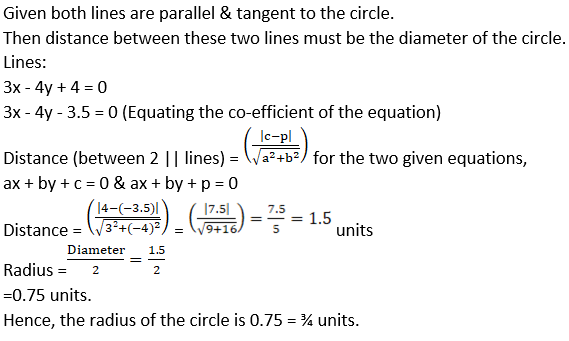6. Find the equation of a circle which touches both the axes and the line 3x – 4y + 8 = 0 and lies in the third quadrant.

Solution: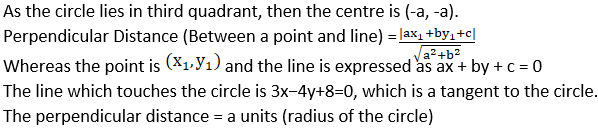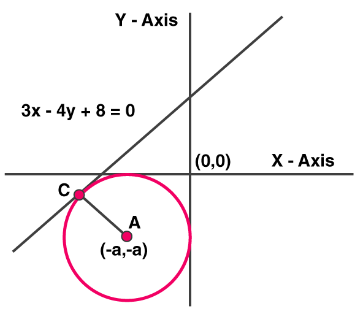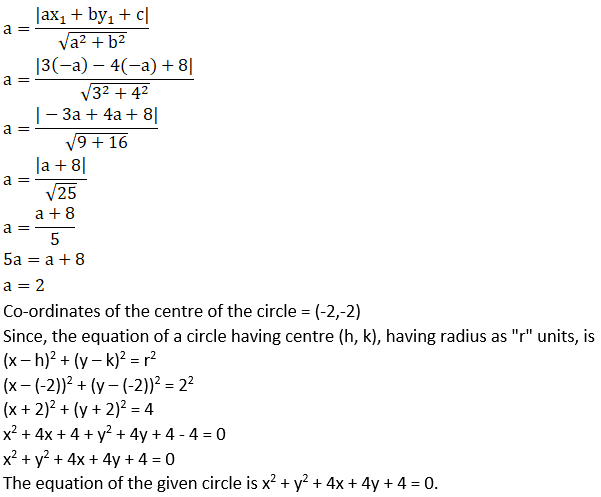The equation of the given circle is x2 + y2 + 4x + 4y + 4 = 0.

7. If one end of a diameter of the circle x2 + y2 – 4x – 6y + 11 = 0 is (3, 4), then find the coordinate of the other end of the diameter.

Solution:

Given equation of the circle,

x2 – 4x + y2 – 6y + 11 = 0

x2 – 4x + 4 + y2 – 6y + 9 +11 – 13 = 0

the above equation can be written as

x2 – 2 (2) x + 22 + y2 – 2 (3) y + 32 +11 – 13 = 0

on simplifying we get

(x – 2)2 + (y – 3)2 = 2

(x – 2)2 + (y – 3)2 = (√2)2

Since, the equation of a circle having centre (h, k), having radius as r units, is

(x – h)2 + (y – k)2 = r2

We have centre = (2, 3)

The centre point is the mid-point of the two ends of the diameter of a circle.

Let the points be (p, q)

(p + 3)/2 = 2 and (q + 4)/2 = 3

p + 3 = 4 & q + 4 = 6

p = 1 & q = 2

Hence, the other ends of the diameter are (1, 2).

8. Find the equation of the circle having (1, –2) as its centre and passing through 3x + y = 14, 2x + 5y = 18

Solution:

Solving the given equations,

3x + y = 14 ……….1

2x + 5y = 18 …………..2

Multiplying the first equation by 5, we get

15x + 5y = 70…….3

2x + 5y = 18……..4

Subtract equation 4 from 3 we get

13 x = 52,

Therefore x = 4

Substituting x = 4, in equation 1, we get

3 (4) + y = 14

y = 14 – 12 = 2

So, the point of intersection is (4, 2)

Since, the equation of a circle having centre (h, k), having radius as r units, is

(x – h)2 + (y – k)2 = r2

Putting the values of (4, 2) and centre co-ordinates (1,-2) in the above expression, we get

(4 – 1)2 + (2 – (-2))2 = r2

32 + 42 = r2

r2 = 9 + 16 = 25

r = 5 units

So, the expression is

(x – 1)2 + (y – (-2))2 = 52

Expanding the above equation we get

x2 – 2x + 1 + (y + 2)2 = 25

x2 – 2x + 1 + y2 + 4y + 4 = 25

x2 – 2x + y2 + 4y – 20 = 0

Hence the required expression is x2 – 2x + y2 + 4y – 20 = 0.

9. If the line y = √3x + k touches the circle x2 + y2 = 16, then find the value of k.

Solution: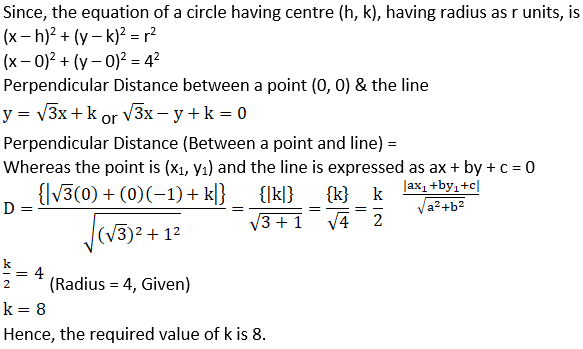Hence, the required value of k is 8.

10. Find the equation of a circle concentric with the circle x2 + y2 – 6x + 12y + 15 = 0 and has double of its area.

Solution:

Given equation of the circle is

x2 – 6x + y2 + 12y + 15 = 0

The above equation can be written as

x2 – 2 (3) x + 32 + y2 + 2 (6) y + 62 + 15 – 9 + 36 = 0

(x – 3)2 + (y-(-6))2 – 30 = 0

(x – 3)2 + (y-(-6))2 = (√30)2

Since, the equation of a circle having centre (h, k), having radius as r units, is

(x – h)2 + (y – k)2 = r2

Centre = (3,-6)

Area of inner circle = πr2 = 22/7 × 30 = 30 π units square

Area of outer circle = 2 × 30 π = 60 π units square

So, πr2 = 60 π

r2 = 60

Equation of outer circle is,

(x – 3)2 + (y – (-6))2 = (√60)2

x2 – 6x + 9 + y2 – 12 y + 36 = 60

x2 – 6x + y2 +12y +45 – 60 = 0

x2 – 6x + y2 + 12y – 15 = 0

Hence, the required equation of the circle is x2 – 6x + y2 + 12y – 15 = 0.

11. If the latus rectum of an ellipse is equal to half of minor axis, then find its eccentricity.

Solution: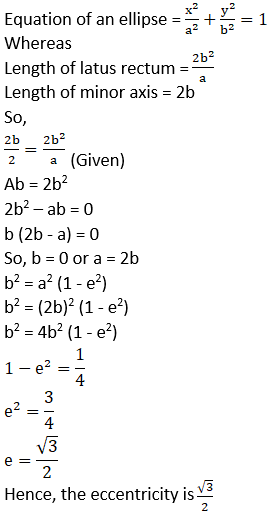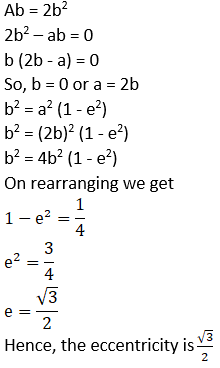12. Given the ellipse with equation 9x2 + 25y2 = 225, find the eccentricity and foci.

Solution: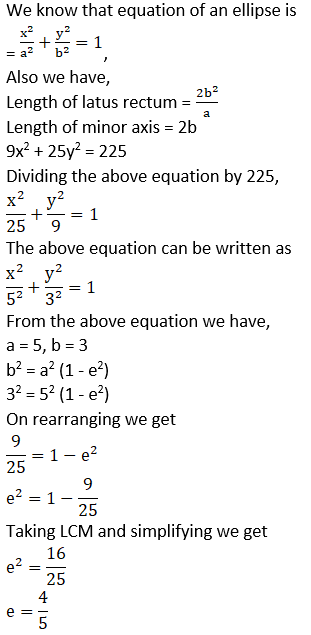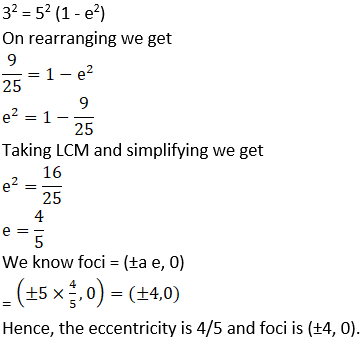13. If the eccentricity of an ellipse is 5/8 and the distance between its foci is 10, then find latus rectum of the ellipse.

Solution: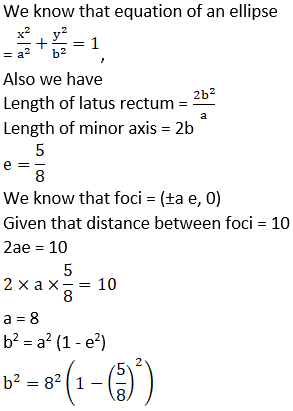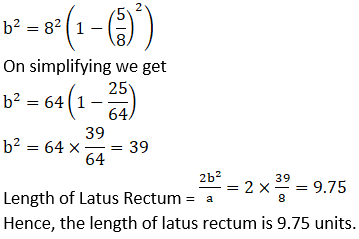14. Find the equation of ellipse whose eccentricity is 2/3 , latus rectum is 5 and the centre is (0, 0).

Solution: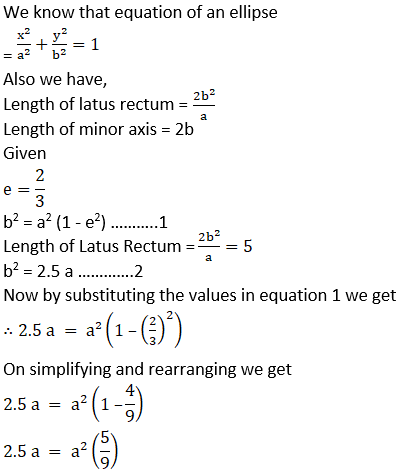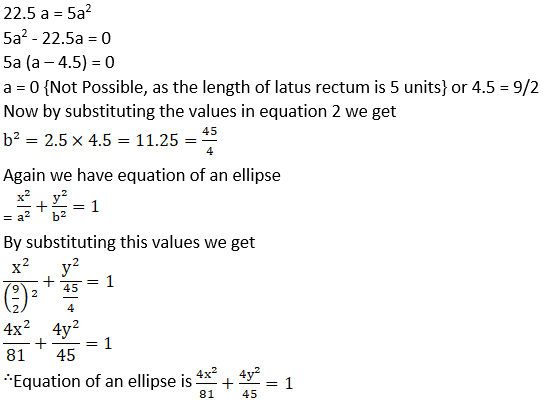15. Find the distance between the directrices of the ellipse x2/36 + y2/20 = 1

Solution: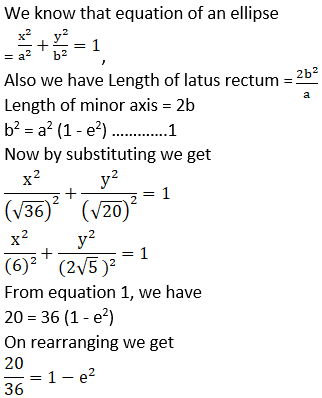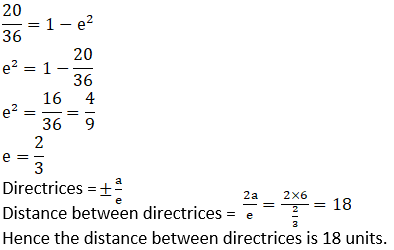16. Find the coordinates of a point on the parabola y2 = 8x whose focal distance is 4.

Solution:

We know that equation of an ellipse is y2 = 4ax,

Also we have length of latus rectum = 4a

Now by comparing the above two equations,

4a = 8

Therefore

a = 2

y2 = 8 × 2 = 16

y = ± 4 and x = 2

Hence the co – ordinates are (2, 4) and (2, -4).

17. Find the length of the line-segment joining the vertex of the parabola y2 = 4axand a point on the parabola where the line-segment makes an angle q to the x-axis.

Solution: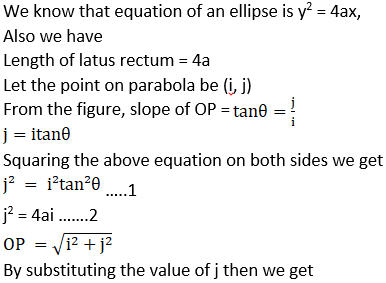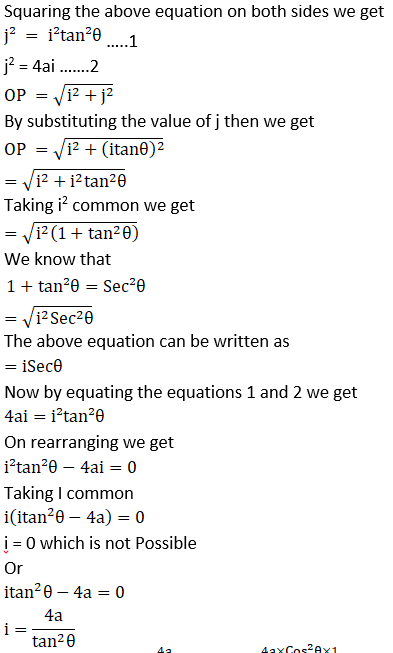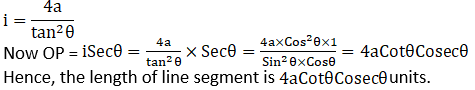18. If the points (0, 4) and (0, 2) are respectively the vertex and focus of a parabola, then find the equation of the parabola.

Solution:

Given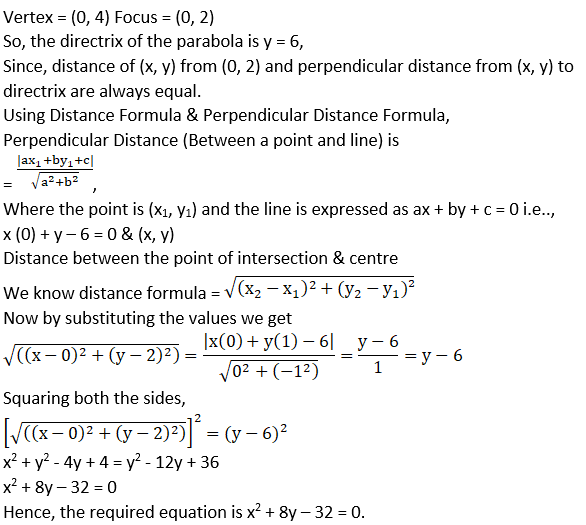19. If the line y = mx + 1 is tangent to the parabola y2 = 4x then find the value of m.

Solution:

Given equations are,

y = mx + 1 & y2 = 4x

By solving given equations we get

(mx + 1)2 = 4x

Expanding the above equation we get

m2x2 + 2mx + 1 = 4x

On rearranging we get

m2x2 + 2mx – 4x + 1 = 0

m+x2 + x (2m – 4) + 1 = 0

As the line touches the parabola, above equation must have equal roots,

Discriminant (D) = 0

(2m – 4)2 – 4 (m2) (1) = 0

4m2 – 16m + 16 – 4m2 = 0

-16 m + 16 = 0

– m + 1 = 0

m = 1

Hence, the required value of m is 1.

20. If the distance between the foci of a hyperbola is 16 and its eccentricity is √2, then obtain the equation of the hyperbola.

Solution: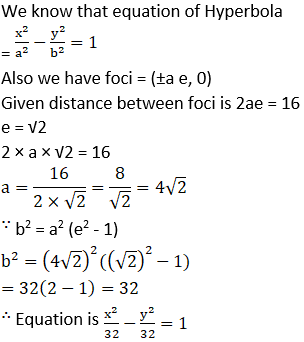21. Find the eccentricity of the hyperbola 9y2 – 4x2 = 36.

Solution: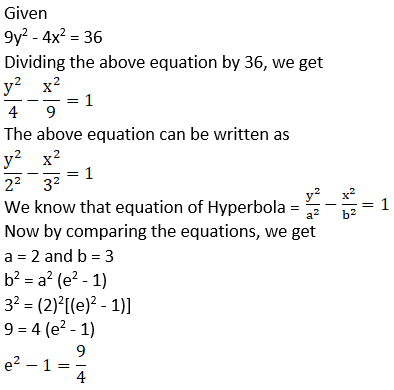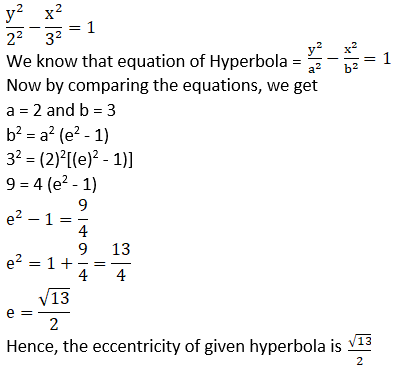22. Find the equation of the hyperbola with eccentricity 3/2 and foci at (± 2, 0).

Solution: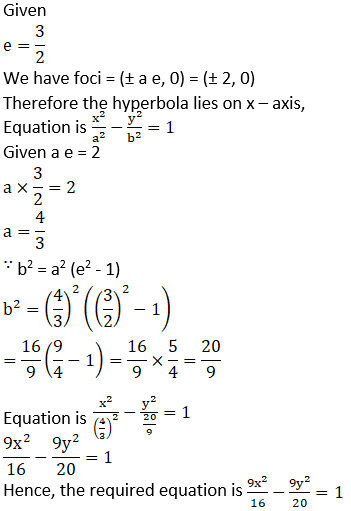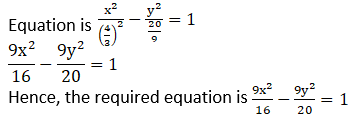23. If the lines 2x – 3y = 5 and 3x – 4y = 7 are the diameters of a circle of area 154square units, then obtain the equation of the circle.

Solution:

Since, diameters of a circle intersect at the centre of a circle,

2x – 3y = 5 ………1

3x – 4y = 7 ………..2

Solving the above equations,

Multiplying equation 1 by 3 we get

6x – 9y = 15

Multiplying equation 2 by 2 we get

6x – 8y = 14

y = 1

y = -1

Putting y = -1, in equation 1, we get

2x – 3(-1) = 5

2x + 3 = 5

2x = 2

x = 1

Coordinates of centre = (1,-1)

Given area = 154

Area = πr2 = 154

22/7 × r2 = 154

r2 = 154 × 7/22

r = 7 units

Since, the equation of a circle having centre (h, k), having radius as r units, is

(x – h)2 + (y – k)2 = r2

(x – 1)2 + (y – (-1))2 = 72

x2 – 2x +1 + (y + 1)2 = 49

x2 – 2x + 1 + y2 + 2y + 1 – 49 = 0

x2 – 2x + y2 + 2y – 47 = 0

Hence the required equation of the given circle is x2 – 2x + y2 + 2y – 47 = 0.

24. Find the equation of the circle which passes through the points (2, 3) and (4, 5) and the centre lies on the straight line y – 4x + 3 = 0.

Solution:

Since, the equation of a circle having centre (h, k), having radius as r units, is

(x – h)2 + (y – k)2 = r2………..1

Substituting (2, 3) & (4, 5) in the above equation, we get

(2 – h)2 + (3 – k)2 = r2

4 – 4h + h2 + 9 + k2 – 6k = r2

h2 – 4h + k2 – 6k + 13 = r2 …………..2

(4 – h)2 + (5 – k)2 = r2

16 – 8h + h2 + 25 + k2 – 10k = r2

h2 – 8h + k2 – 10k + 41 = r2 ………..3

Equating both the equations 2 & 3, as their RHS are equal, we get

h2 – 4h + k2 – 6k + 13 = h2 – 8h + k2 – 10k + 41

On simplifying we get

8h – 4h + 10k – 6k = 41 – 13

4h + 4k = 28

h + k = 7 ………..4

As centre lies on the given line, so it satisfies the values too,

k – 4h + 3 = 0…………5

Solving equations 3 and 4 simultaneously,

h + k = 7

-4h + k = -3

Subtracting both the equations, we get

5h = 10

h = 2

2 + k = 7

k = 5

Putting h = 2 & k = 5 in equation 2,

h2 – 4h + k2 – 6k + 13 = r2

22 – 4(2) + 52 – 6(5) + 13 = r2

4 – 8 + 25 – 30 + 13 = r2

r2 = 4

r = 2 units

Putting the values of h = 2, k = 5 & r = 2, respectively in equation 1,

(x – h)2 + (y – k)2 = r2

(x – 2)2 + (y – 5)2 = 22

x2 – 4x + 4 + y2 – 10y + 25 = 4

x2 – 4x + y2 – 10y + 25 =0

Hence, the required equation is x2 – 4x + y2 – 10y + 25 = 0.

25. Find the equation of a circle whose centre is (3, –1) and which cuts off a chord of length 6 units on the line 2x – 5y + 18 = 0.

Solution: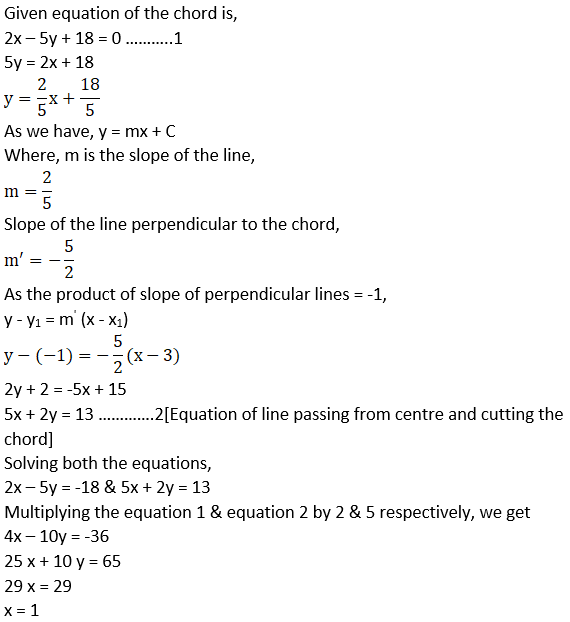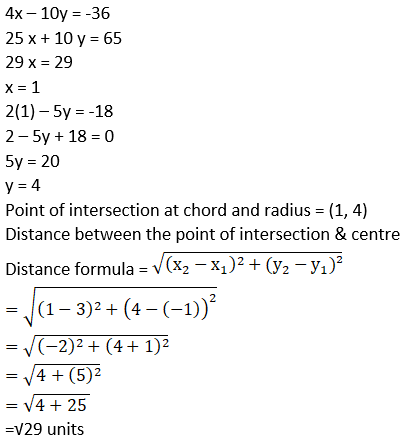Using Pythagoras Theorem,

(Hypotenuse)2 = (Base)2 + (Perpendicular)2

= (3)2 + (√29)2 = 29 + 9

= √38

Since, the radius bisects the chord into two equal halves,

Since, the equation of a circle having centre (h, k), having radius as r units, is

(x – h)2 + (y – k)2 = r2

(x – 3)2 + (y – (-1))2 = (√38)2

x2 – 6x + 9 + (y + 1)2 = 38

x2 – 6x + y2 + 2y + 1 + 9- 38 = 0

x2 – 6x + y2 + 2y – 28 = 0

Hence, the required equation of the circle is x2 – 6x + y2 + 2y – 28 = 0.

26. Find the equation of a circle of radius 5 which is touching another circle x2 + y2 – 2x – 4y – 20 = 0 at (5, 5).

Solution:

Given x2 – 2x + y2 – 4y – 20 = 0

x2 – 2x + 1 +y2 – 4y + 4 – 20 – 5 = 0

(x – 1)2 + (y – 2)2 = 25

(x – 1)2 + (y – 2)2 = 52

Since, the equation of a circle having centre (h, k), having radius as r units, is

(x – h)2 + (y – k)2 = r2

Centre = (1, 2)

Point of Intersection = (5, 5)

It intersects the line into 1: 1, as the radius of both the circles is 5 units.

Using Ratio Formula,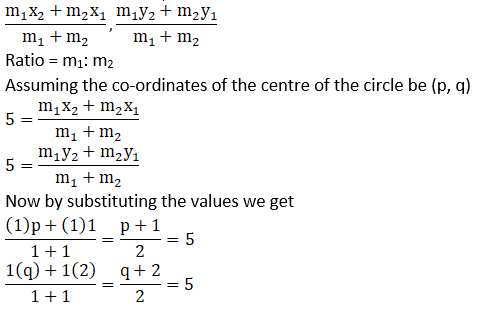p + 1 = 10, q + 2 = 10

p = 9 & q = 8

Co-ordinates = (9, 8)

Therefore the equation is,

(x – h)2 + (y – k)2 = r2

(x – 9)2 + (y – 8)2 = 52

x2 – 18x + 81 + y2 – 16y + 64 = 25

x2 – 18x + y2 – 16y + 145 – 25 = 0

x2 – 18x + y2 – 16y + 120 = 0

Hence, the required equation is x2 – 18x + y2 – 16y + 120 = 0.

27. Find the equation of a circle passing through the point (7, 3) having radius 3 units and whose centre lies on the line y = x – 1.

Solution:

Since, the equation of a circle having centre (h, k), having radius as r units, is

(x – h)2 + (y – k)2 = r2Centre lies on the line i.e., y = x – 1,

Co – Ordinates are (h, k) = (h, h – 1)

(x – h)2 + (y – k)2 = r2

(7 – h)2 + (3 – (h – 1))2 = 32

49 + h2 – 14h + (3 – h +1)2 = 9

On rearranging we get

h2 – 14h + 49 +16 +h2 – 8h – 9 = 0

2h2 – 22h + 56 = 0

h2 – 11h + 28 = 0

h2 – 4h – 7h + 28 = 0

h (h – 4) – 7 (h – 4) = 0

(h – 7) (h – 4) = 0

h = 7 or 4

Centre = (7, 6) or (4, 3)

(x – h)2 + (y – k)2 = r2

Equation, having centre (7, 6)

(x – 7)2 + (y – 6)2 = 32

x2 – 14x + 49 + y2 – 12y + 36 – 9 = 0

x2 – 14x + y2 – 12y + 76 = 0

Equation, having centre (4, 3)

(x – 4)2 + (y – 3)2 = 32

x2 – 8x + 16 + y2 – 6y + 9 – 9 = 0

x2 – 8x + y2 – 6y + 16 = 0

Hence, the required equation is x2 – 14x + y2 – 12y + 76 = 0 or x2 – 8x + y2 – 6y + 16 = 0.

28. Find the equation of each of the following parabolas

(a) Directrix x = 0, focus at (6, 0)

(b) Vertex at (0, 4), focus at (0, 2)

(c) Focus at (–1, –2), directrix x – 2y + 3 = 0

Solution:

(a) The distance of any point on the parabola from its focus and its directrix is same.

Given that, directrix, x = 0 and focus = (6, 0)

If a parabola has a vertical axis, the standard form of the equation of the parabola is (x – h)2 = 4p (y – k), where p≠ 0.

The vertex of this parabola is at (h, k).

The focus is at (h, k + p) & the directrix is the line y = k – p.

As the focus lies on x – axis,

Therefore the equation is y2 = 4ax or y2 = -4ax

So, for any point P(x, y) on the parabola

Distance of point from directrix = Distance of point from focus

x2 = (x – 6)2 + y2

x2 = x2 – 12x + 36 + y2

y2 – 12x + 36 = 0

Hence the required equation is y2 – 12x + 36 = 0.